# NCERT Solutions for Class 6 Maths Chapter 5 – Understanding Elementary Shapes

NCERT Solutions for Class 6 Maths Chapter 5 – Understanding Elementary Shapes comprises of the 9 Exercises

This Chapter contains the Exercises relating to the following topics , which are discussed in Chapter 5 – Understanding Elementary Shapes Class 6 NCERT book  : –

• Exercise 5.1 Introduction
• Exercise 5.2 Measuring Line Segments
• Exercise 5.3 Angles – ‘Right’ and ‘Straight’
• Exercise 5.4 Angles – ‘Acute’, ‘Obtuse’ and ‘Reflex’
• Exercise 5.5 Measuring Angles
• Exercise 5.6 Perpendicular Lines
• Exercise 5.7 Classification of Triangles
• Exercise 5.9 Polygons
• Exercise 5.10 Three Dimensional Shapes

### NCERT Solutions for Class 6 Maths Chapter 5 Exercise 5.1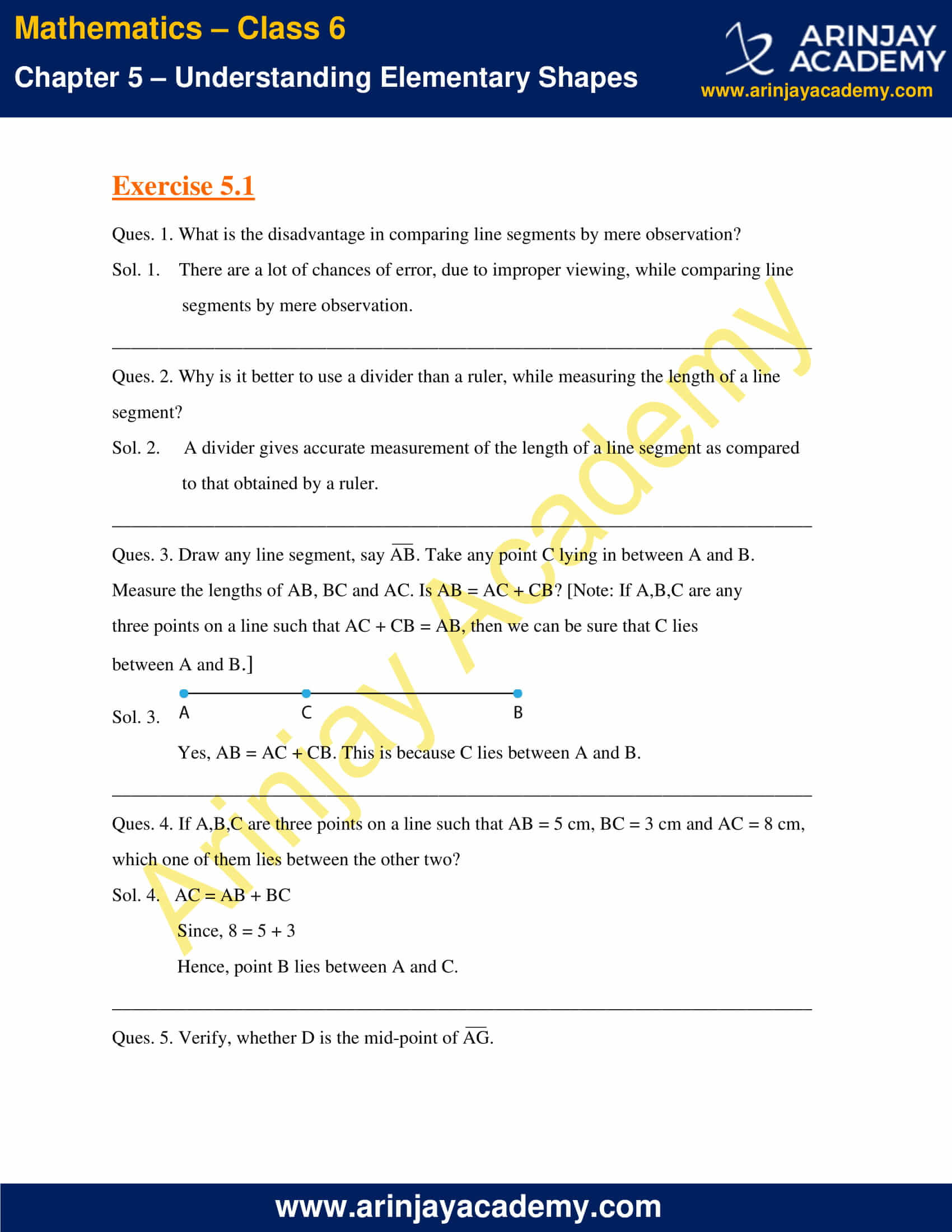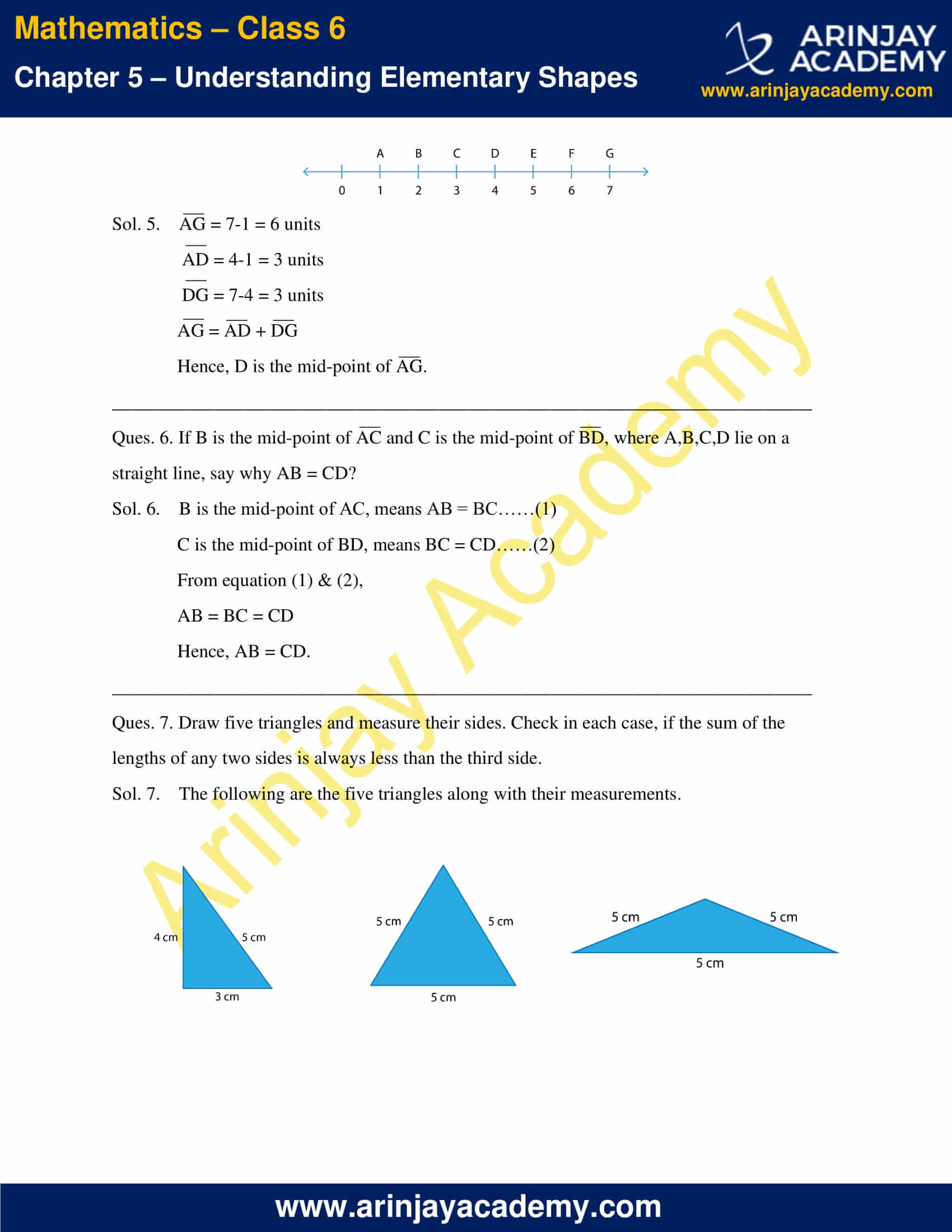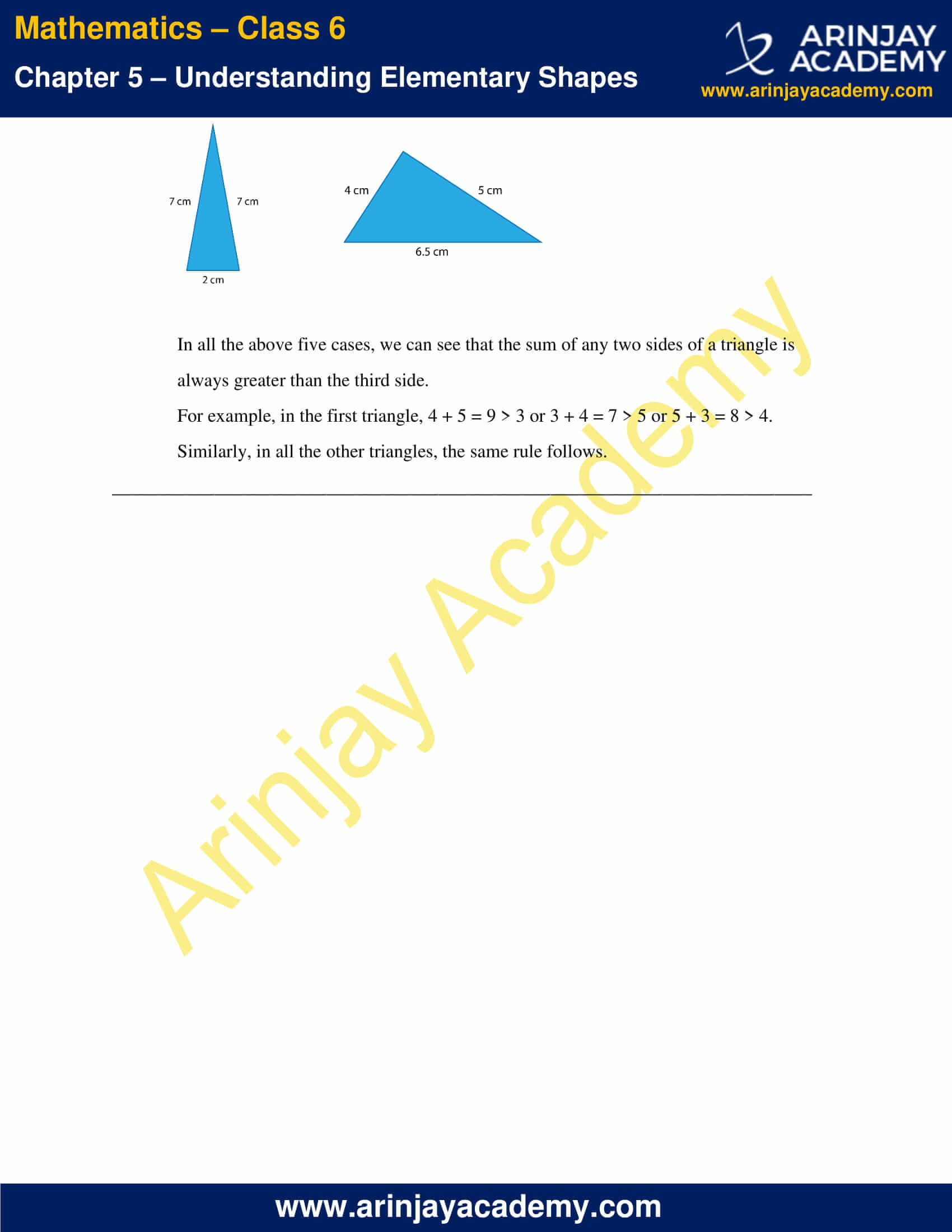### NCERT Solutions for Class 6 Maths Chapter 5 Exercise 5.2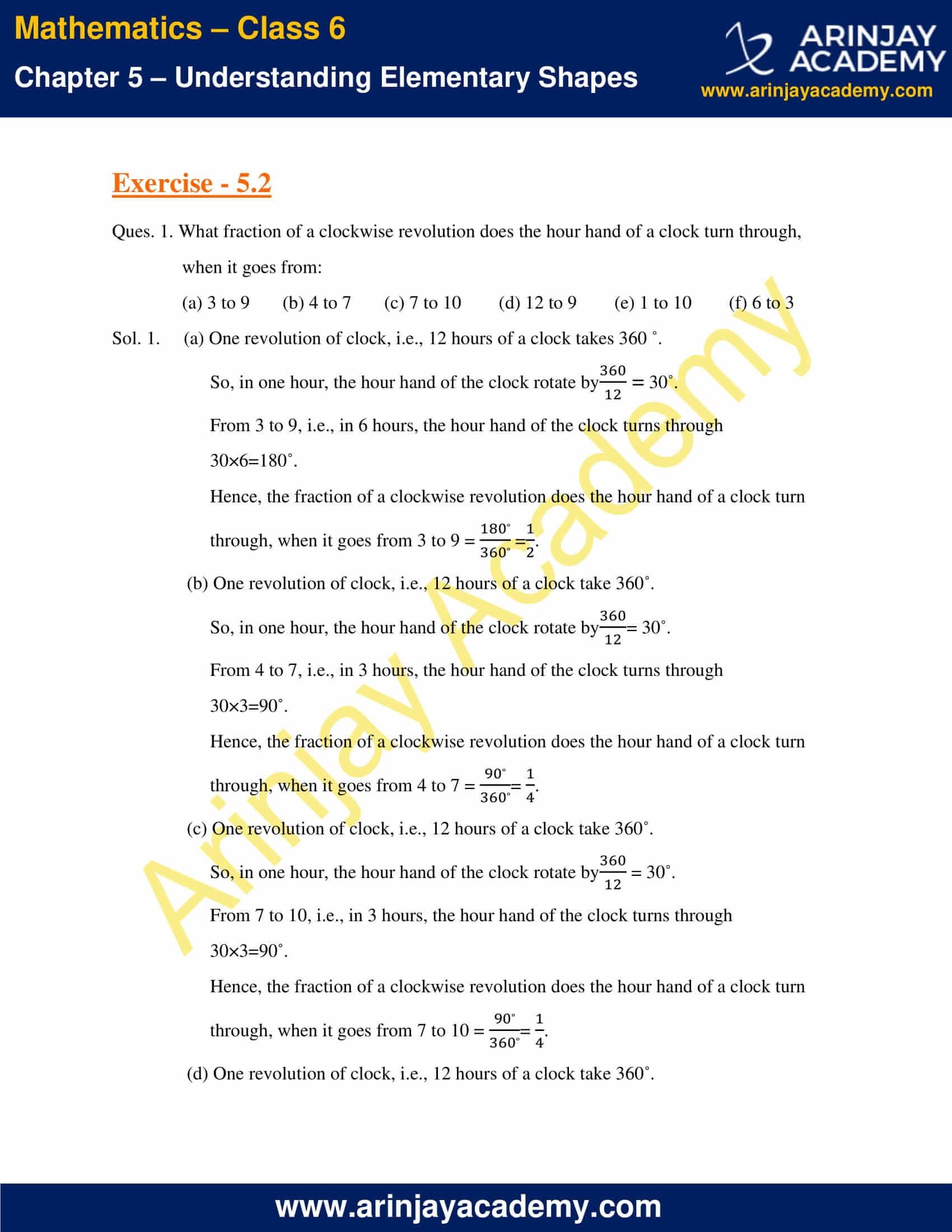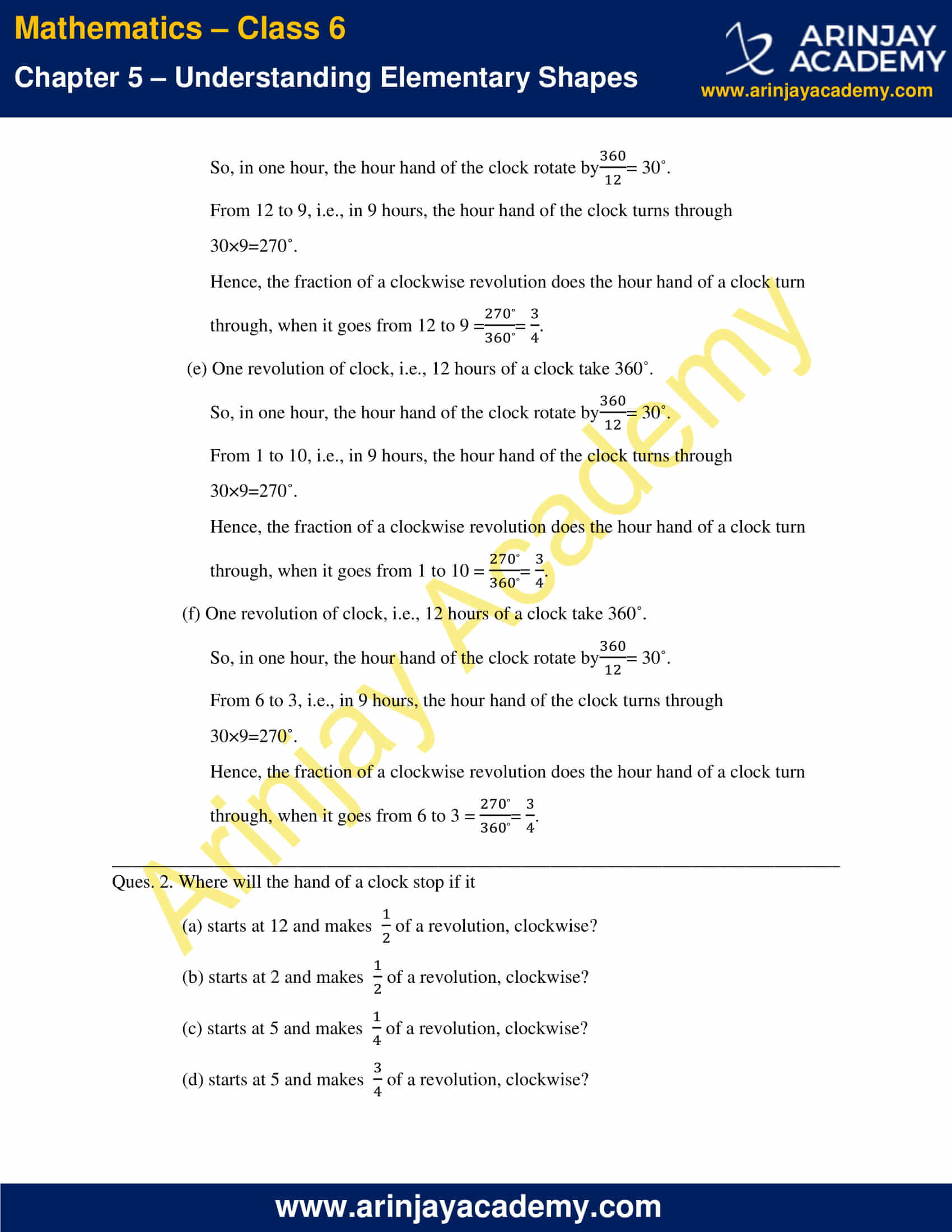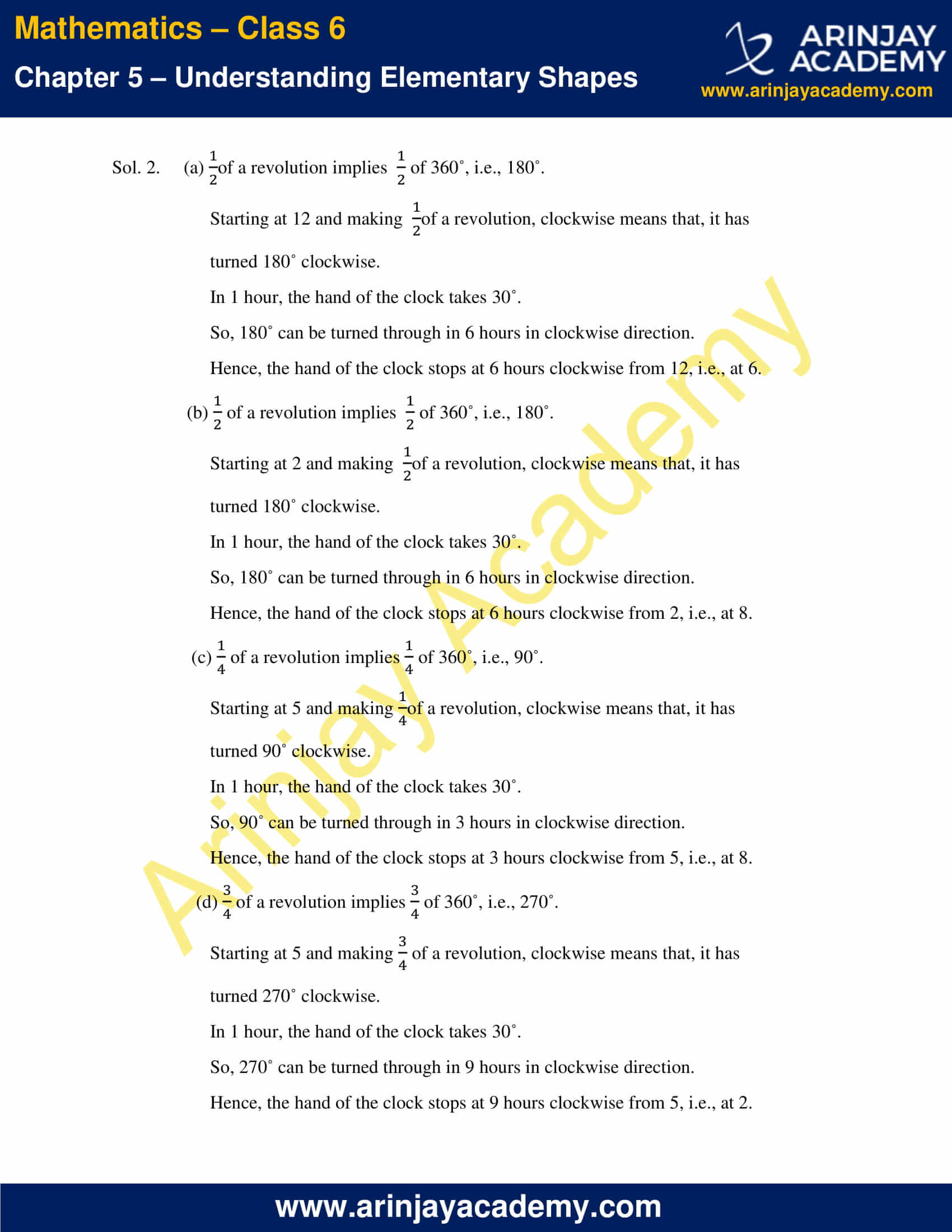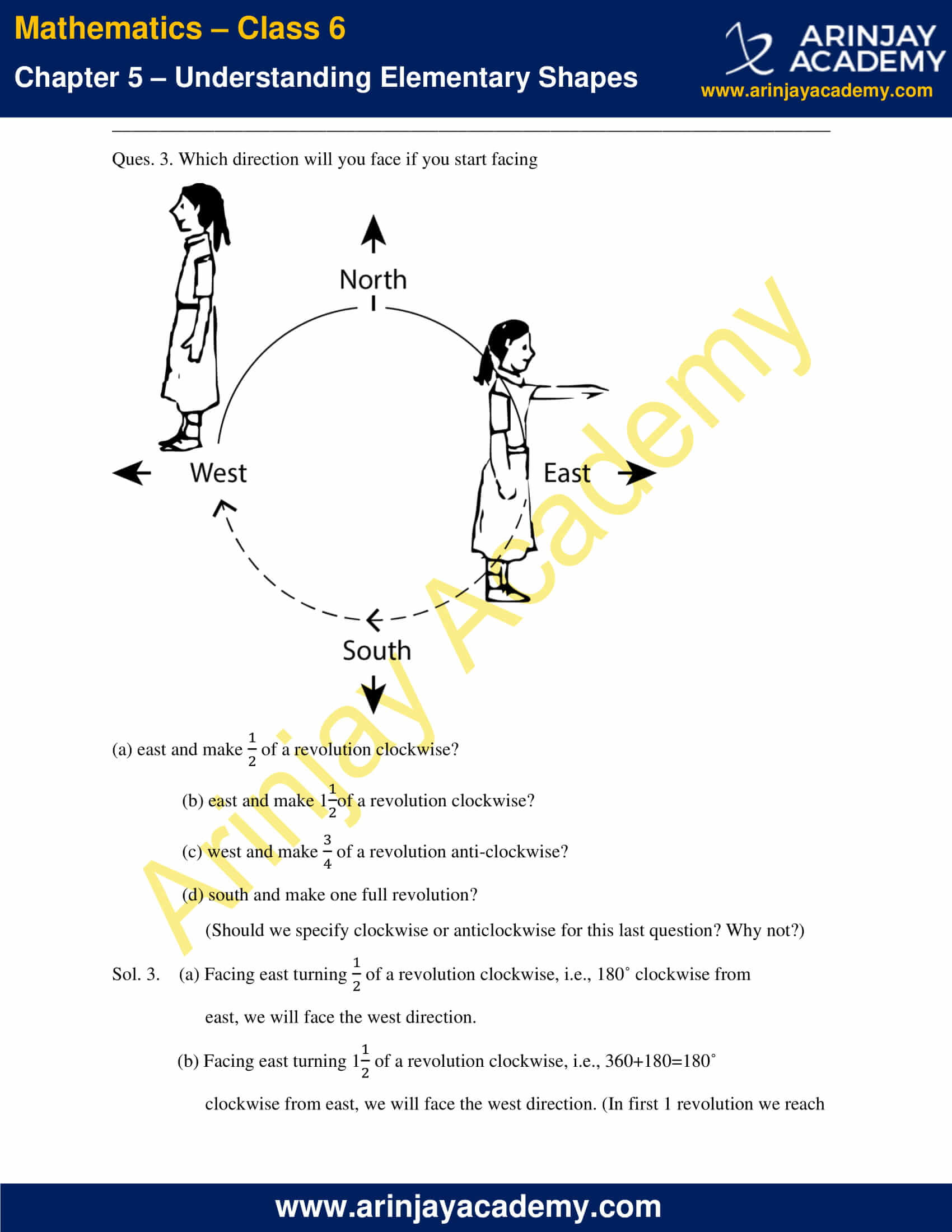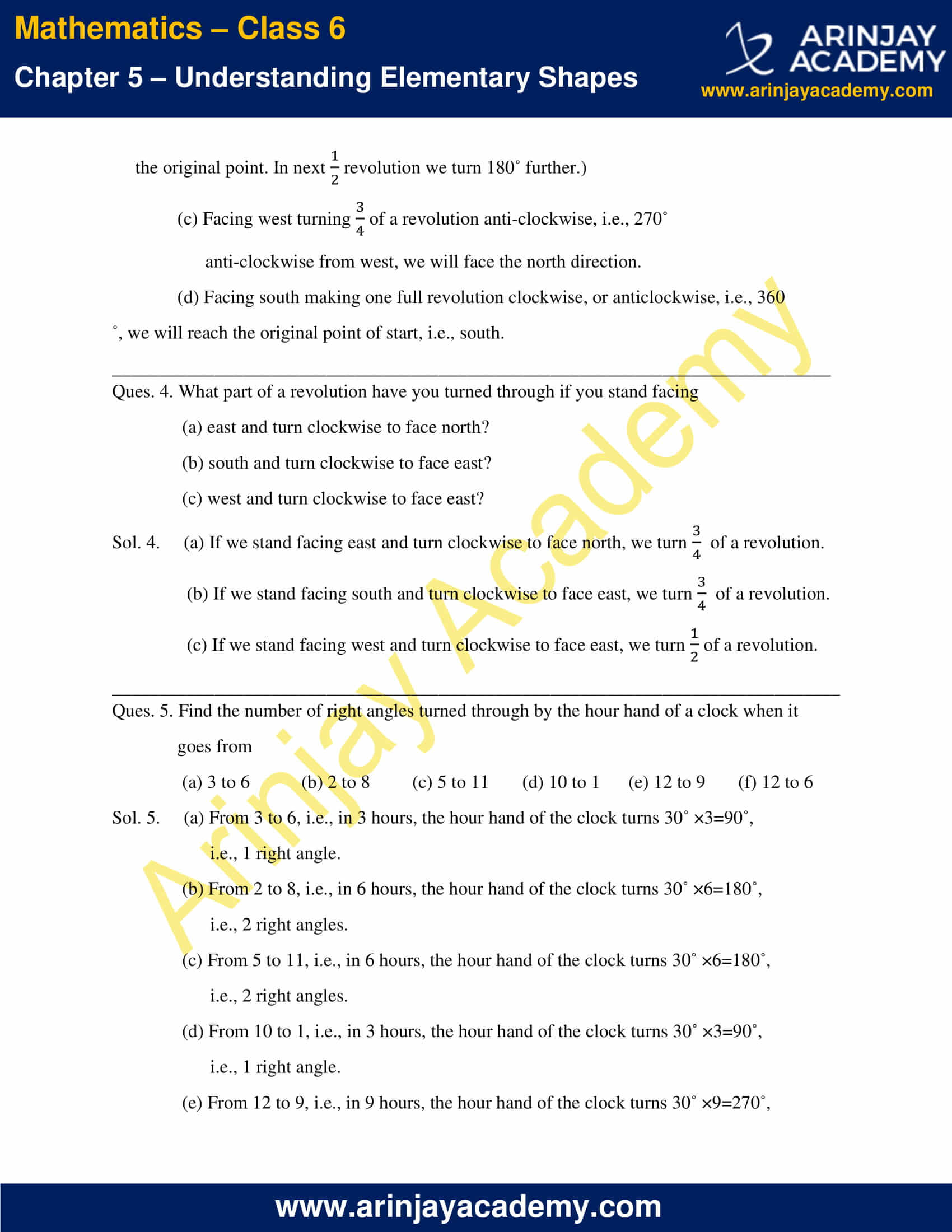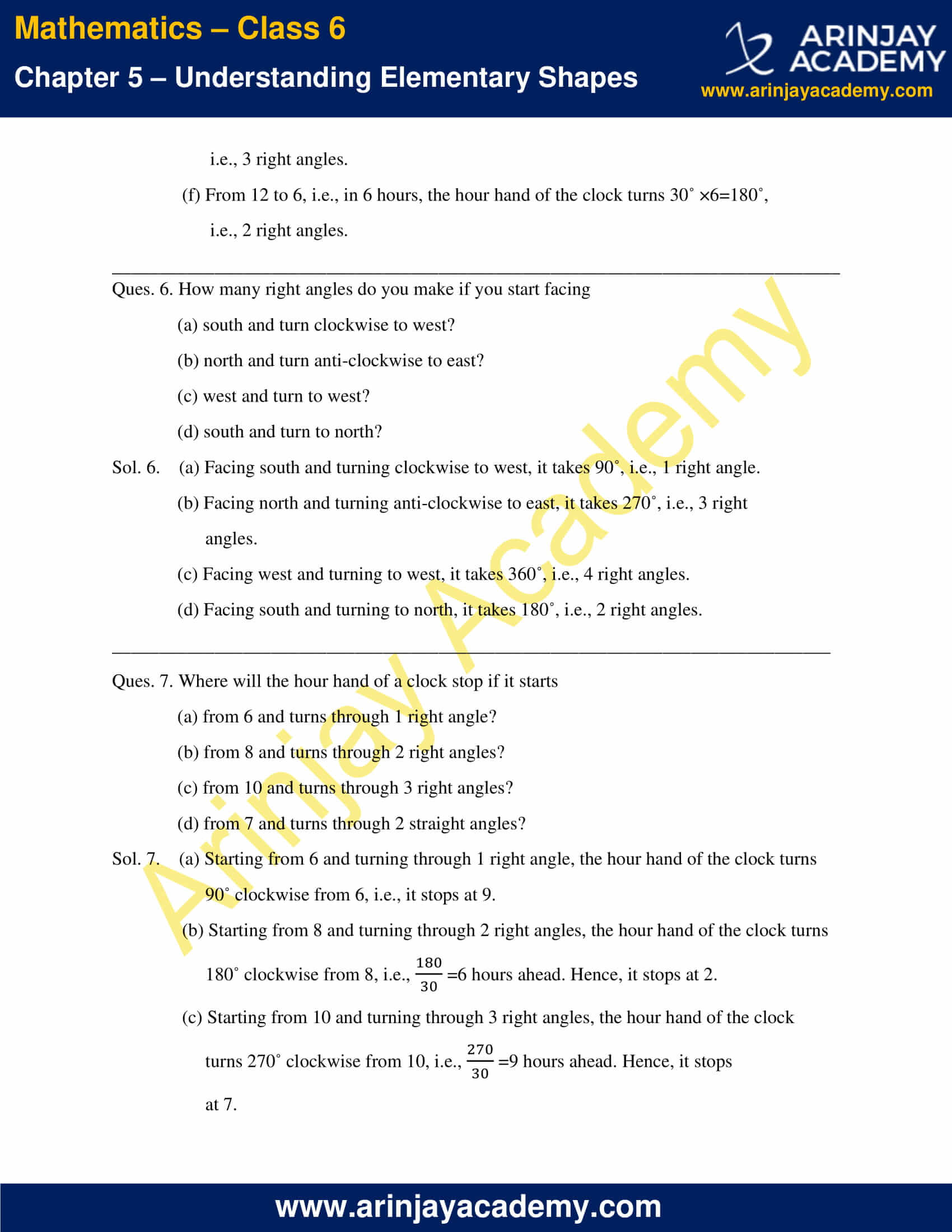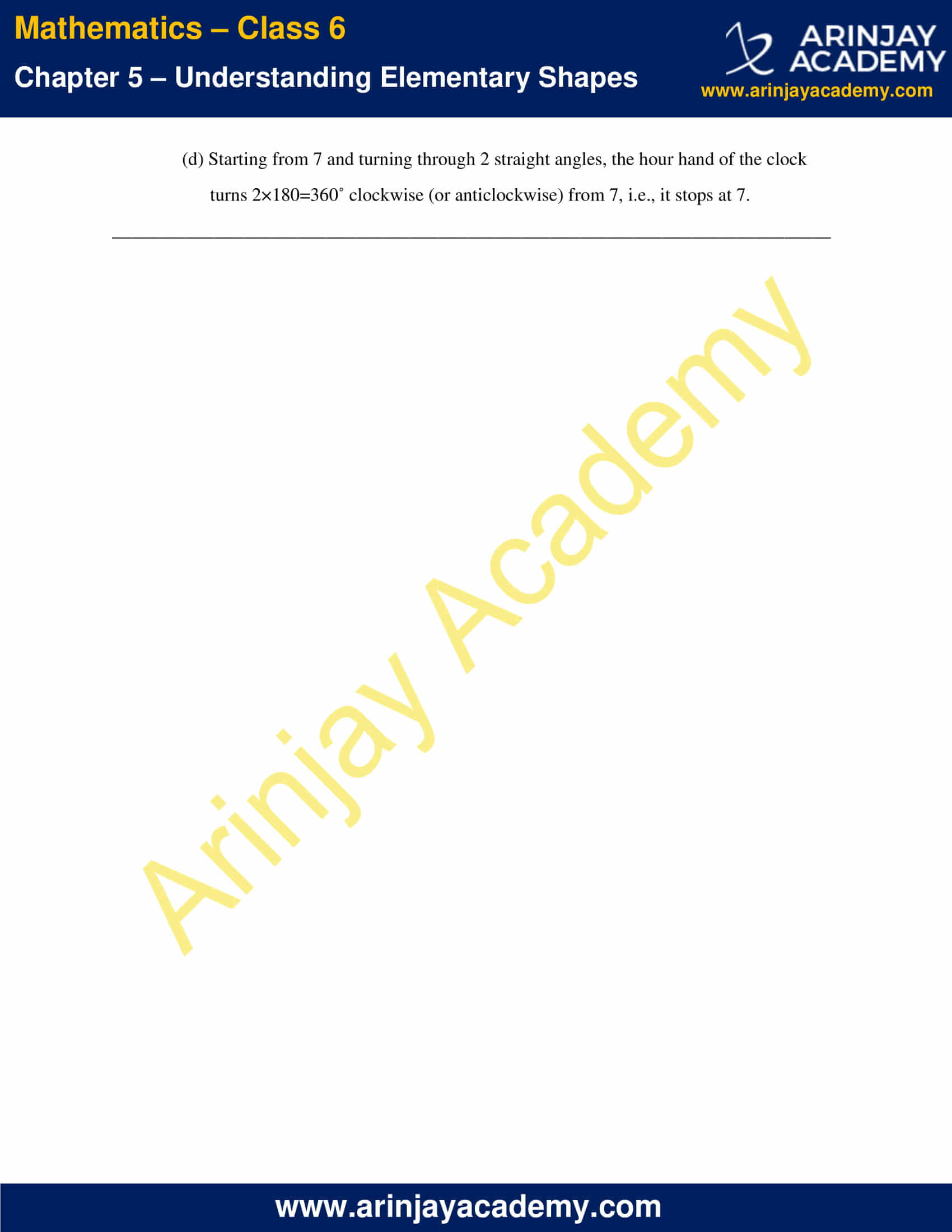### NCERT Solutions for Class 6 Maths Chapter 5 Exercise 5.3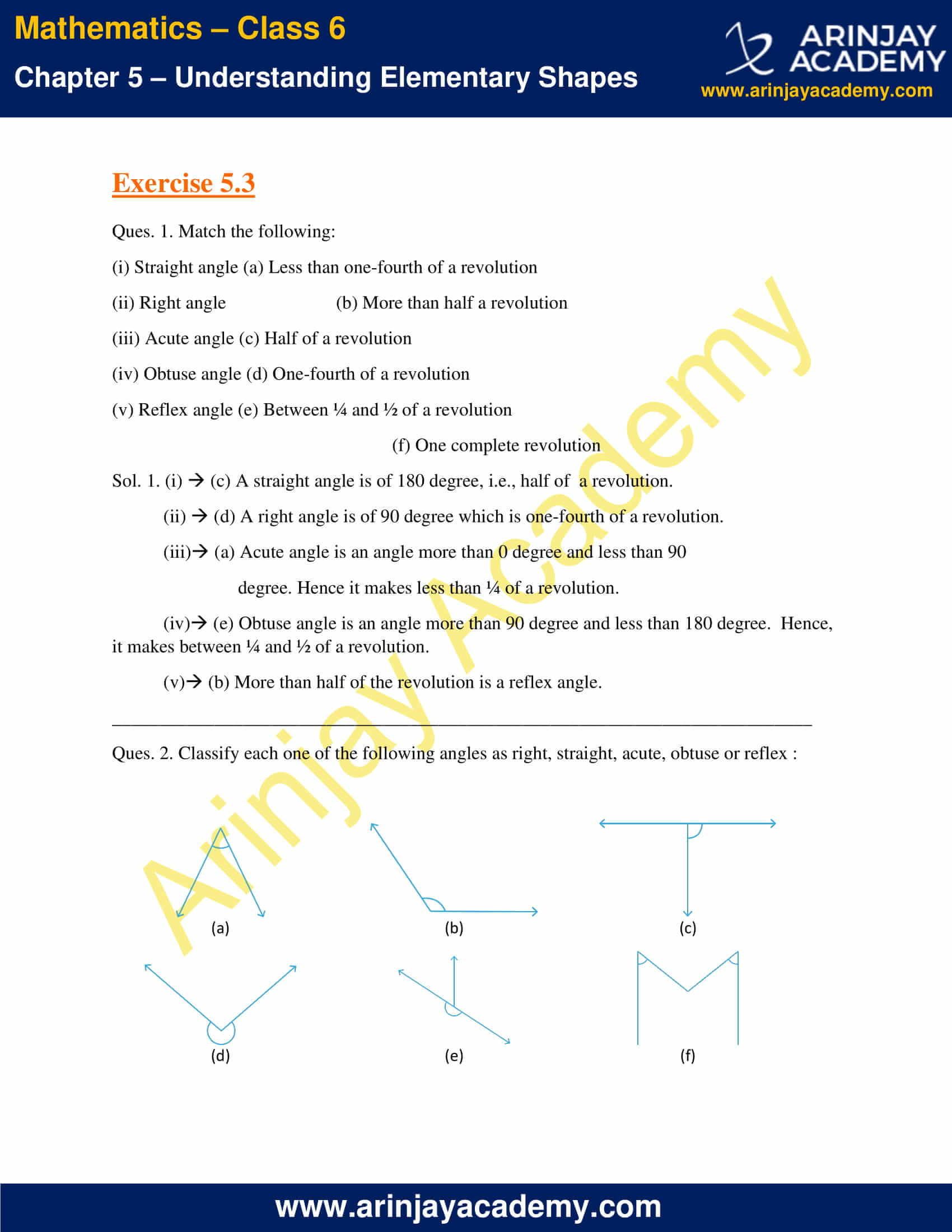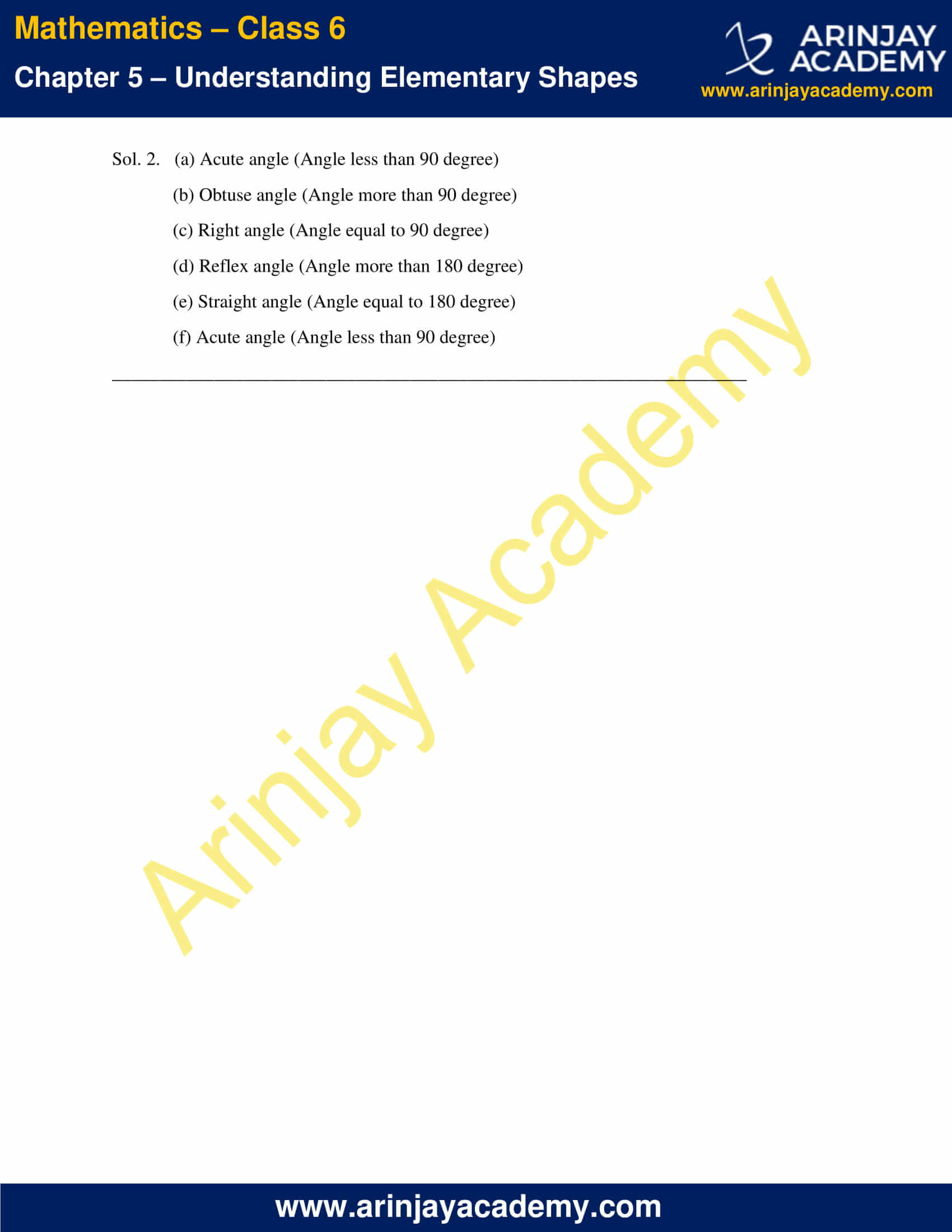### NCERT Solutions for Class 6 Maths Chapter 5 Exercise 5.4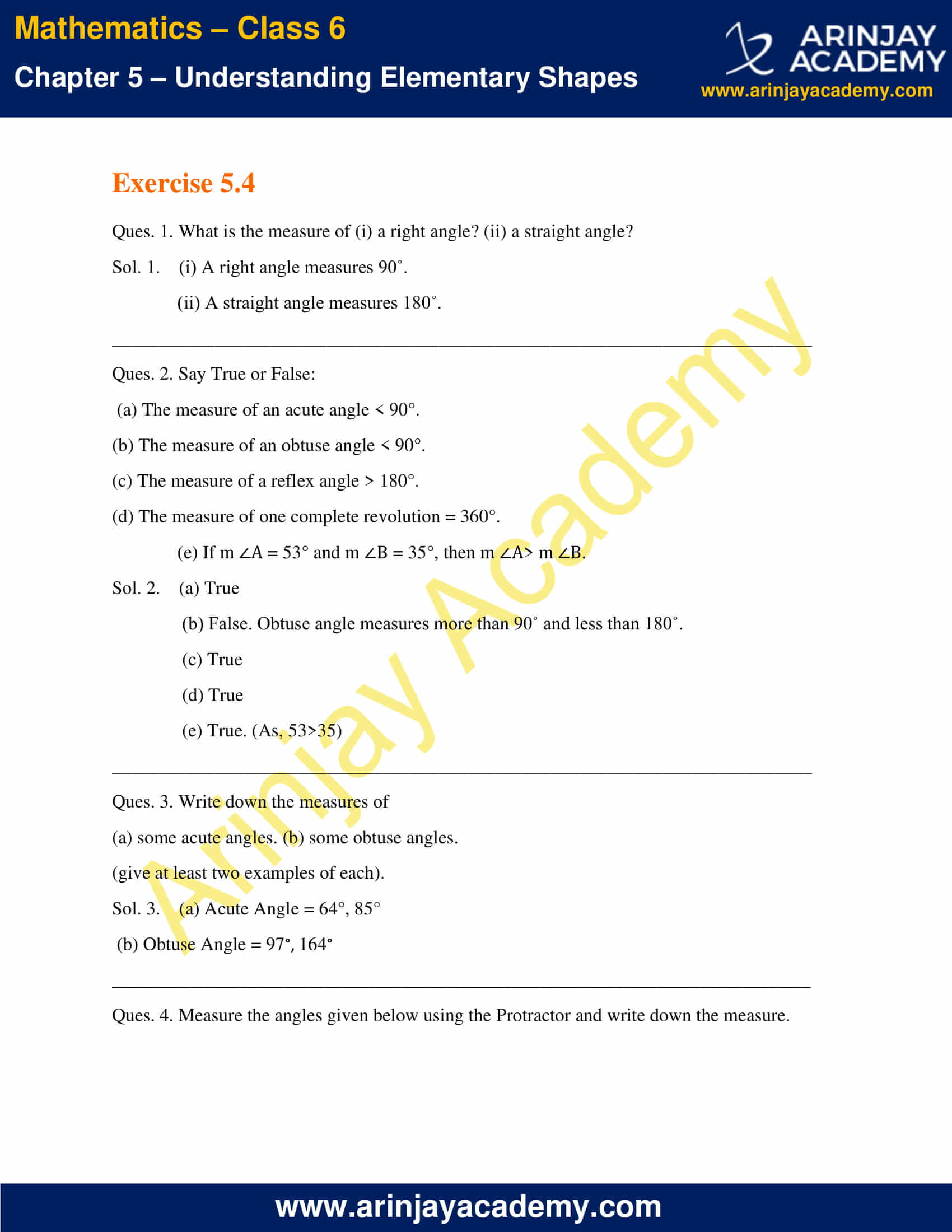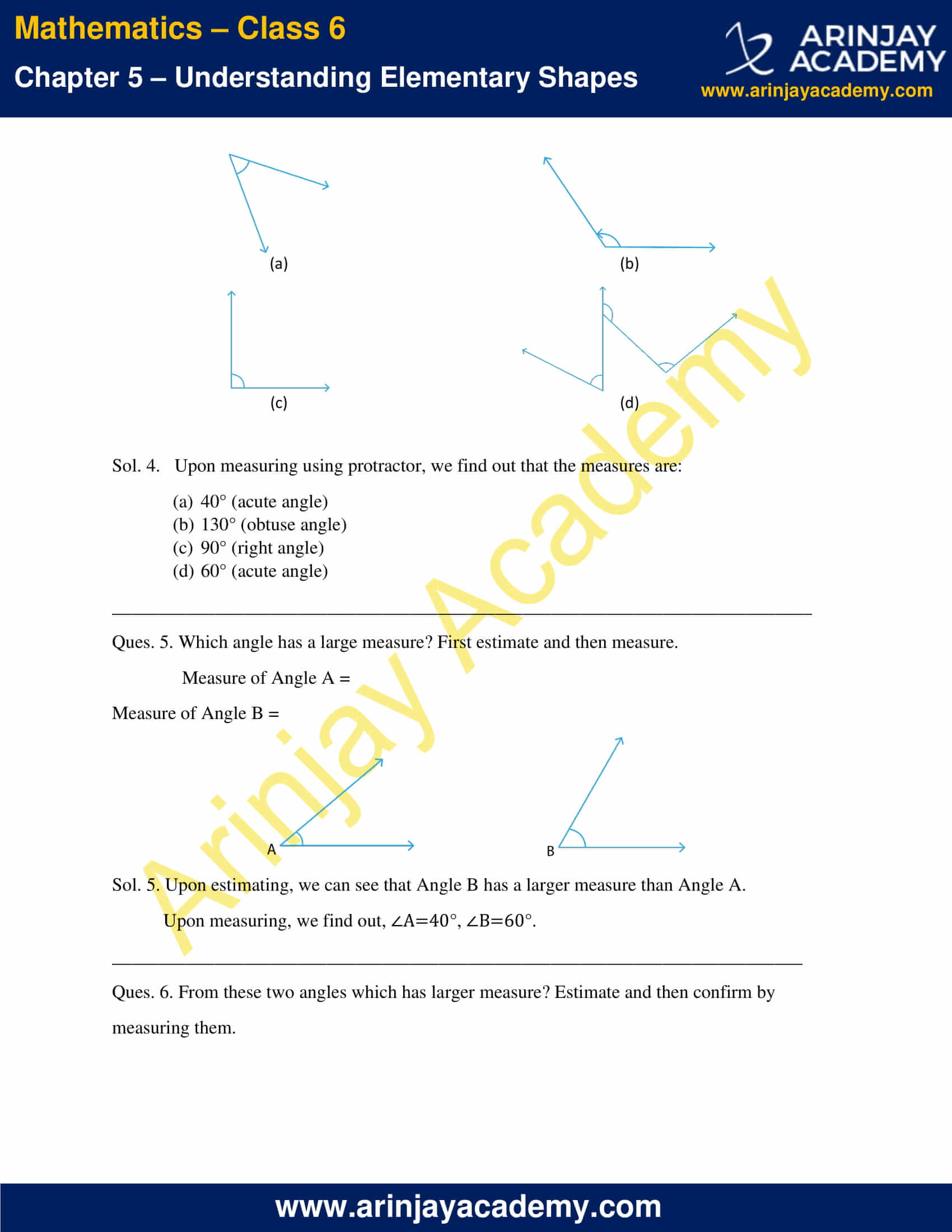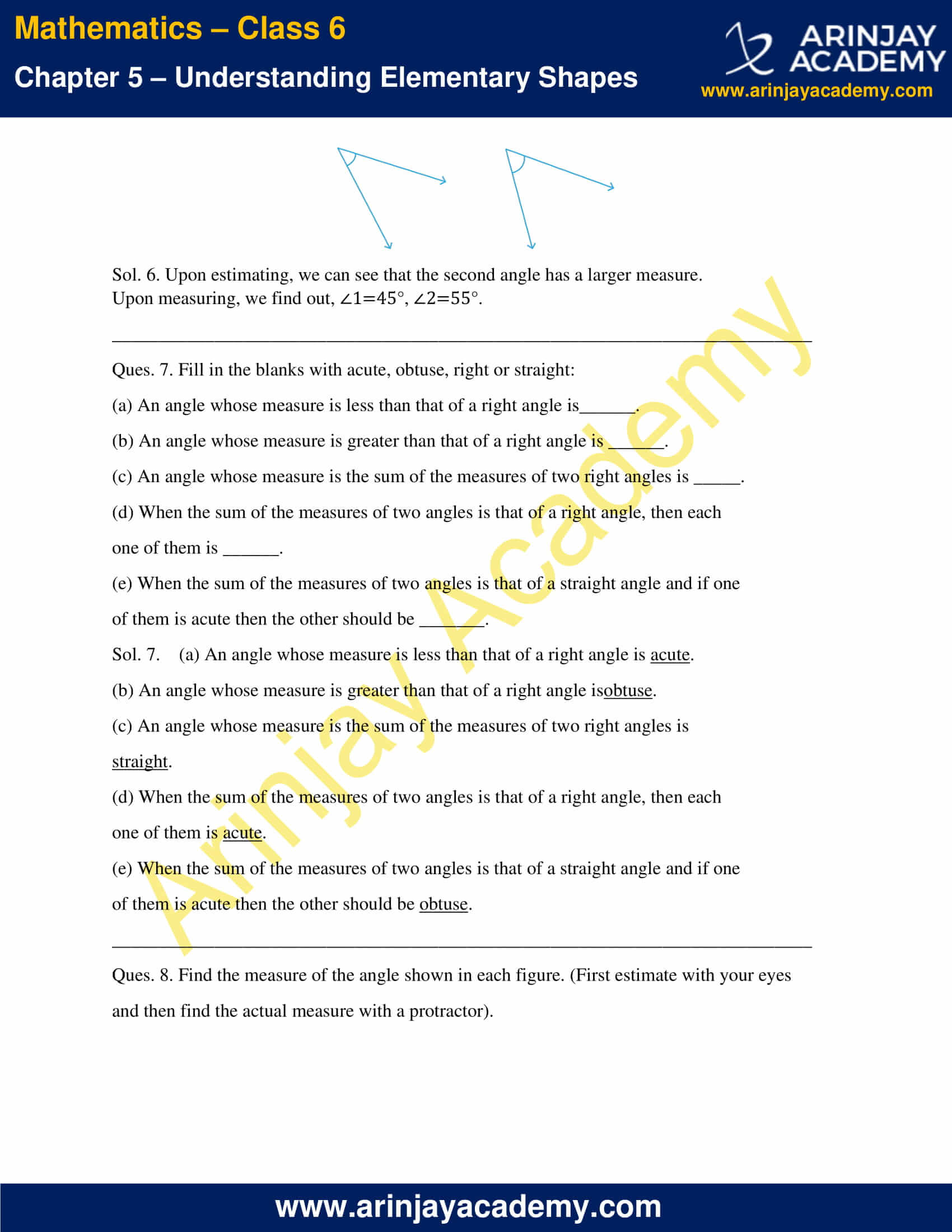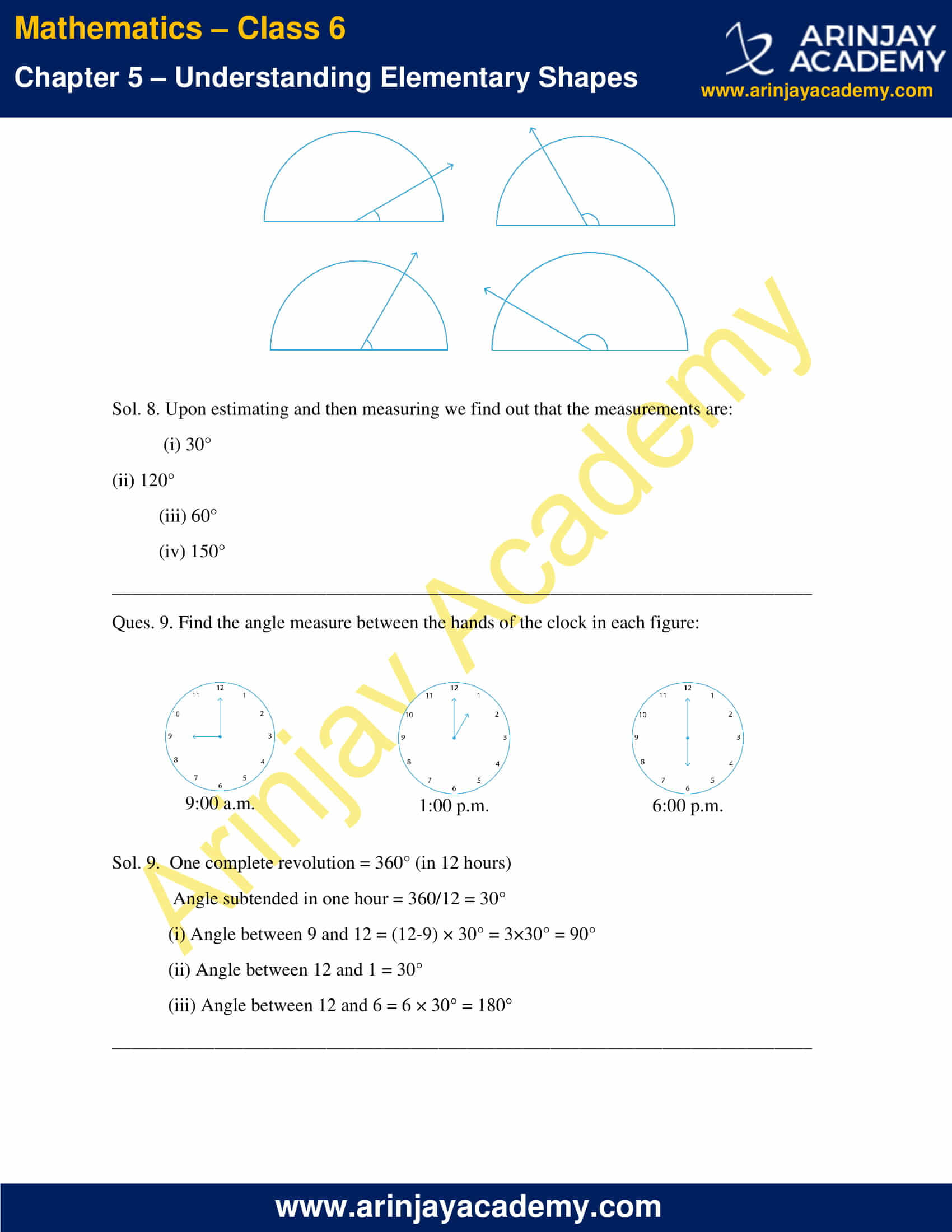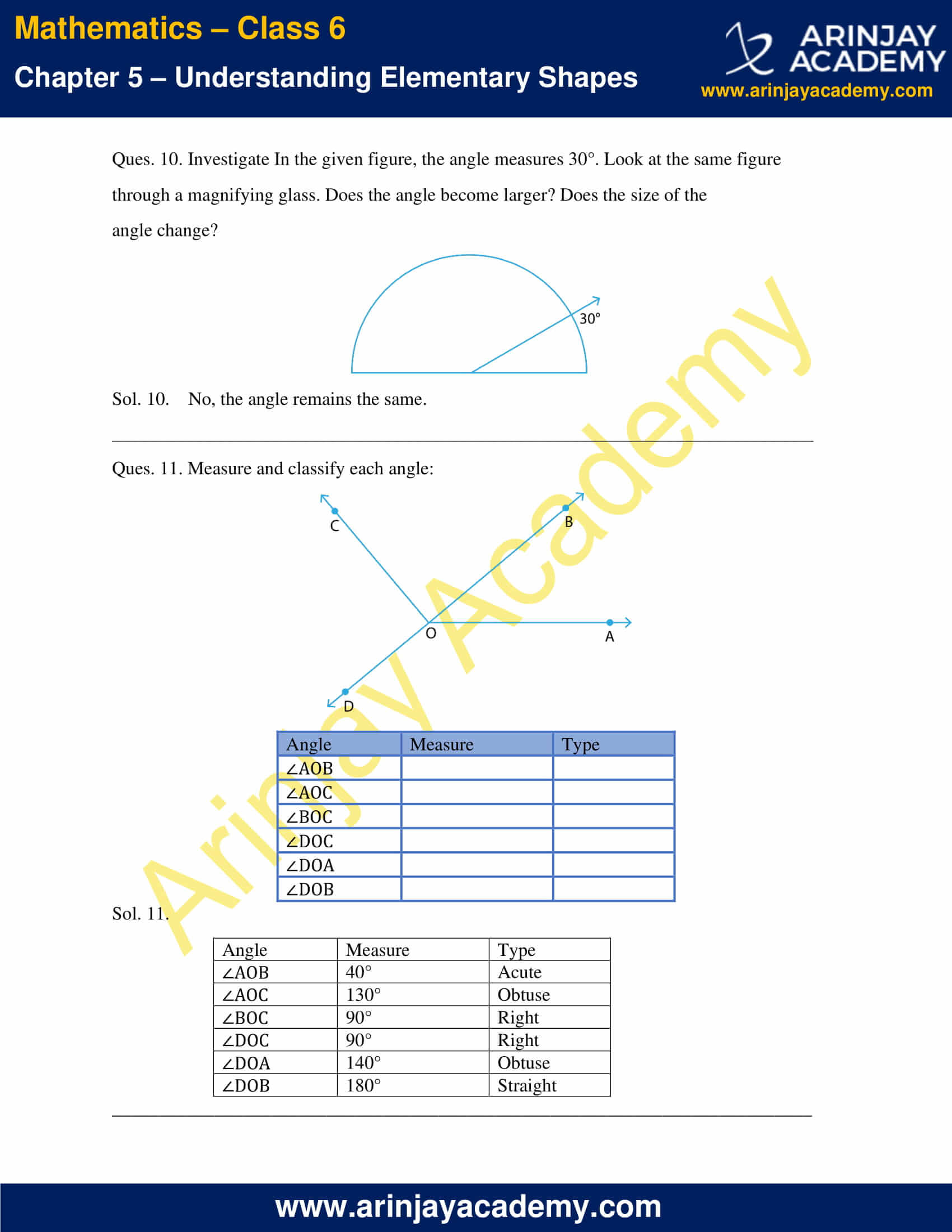### NCERT Solutions for Class 6 Maths Chapter 5 Exercise 5.5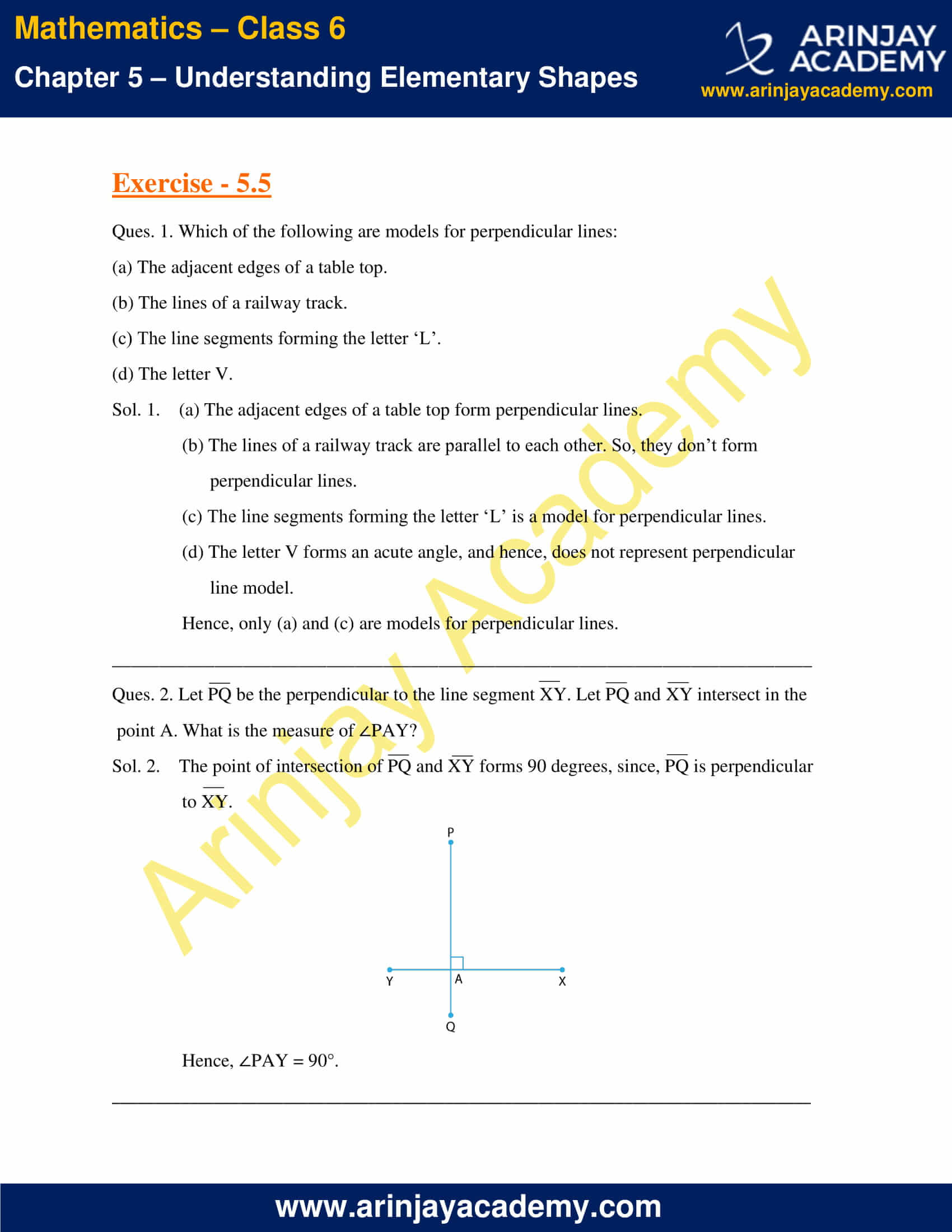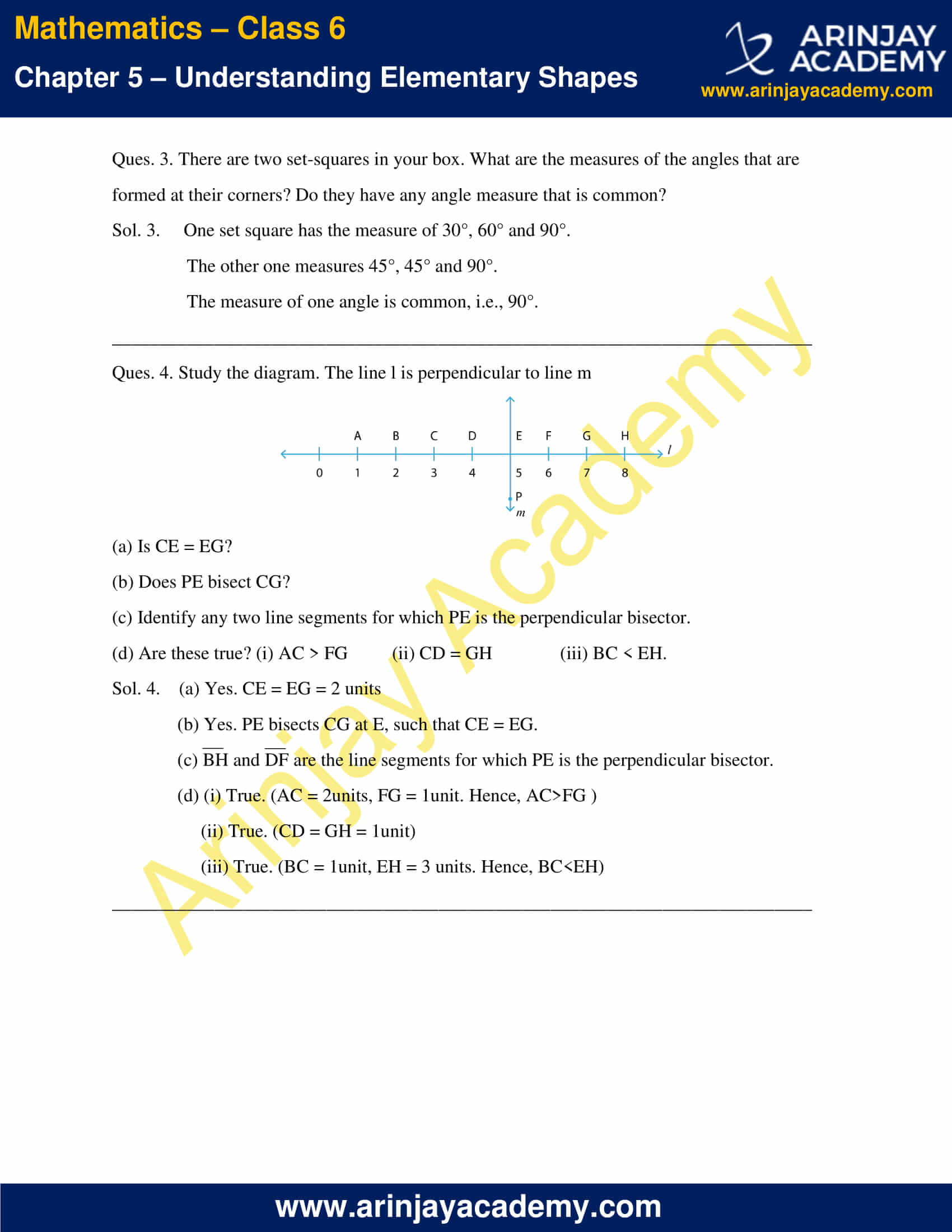### NCERT Solutions for Class 6 Maths Chapter 5 Exercise 5.6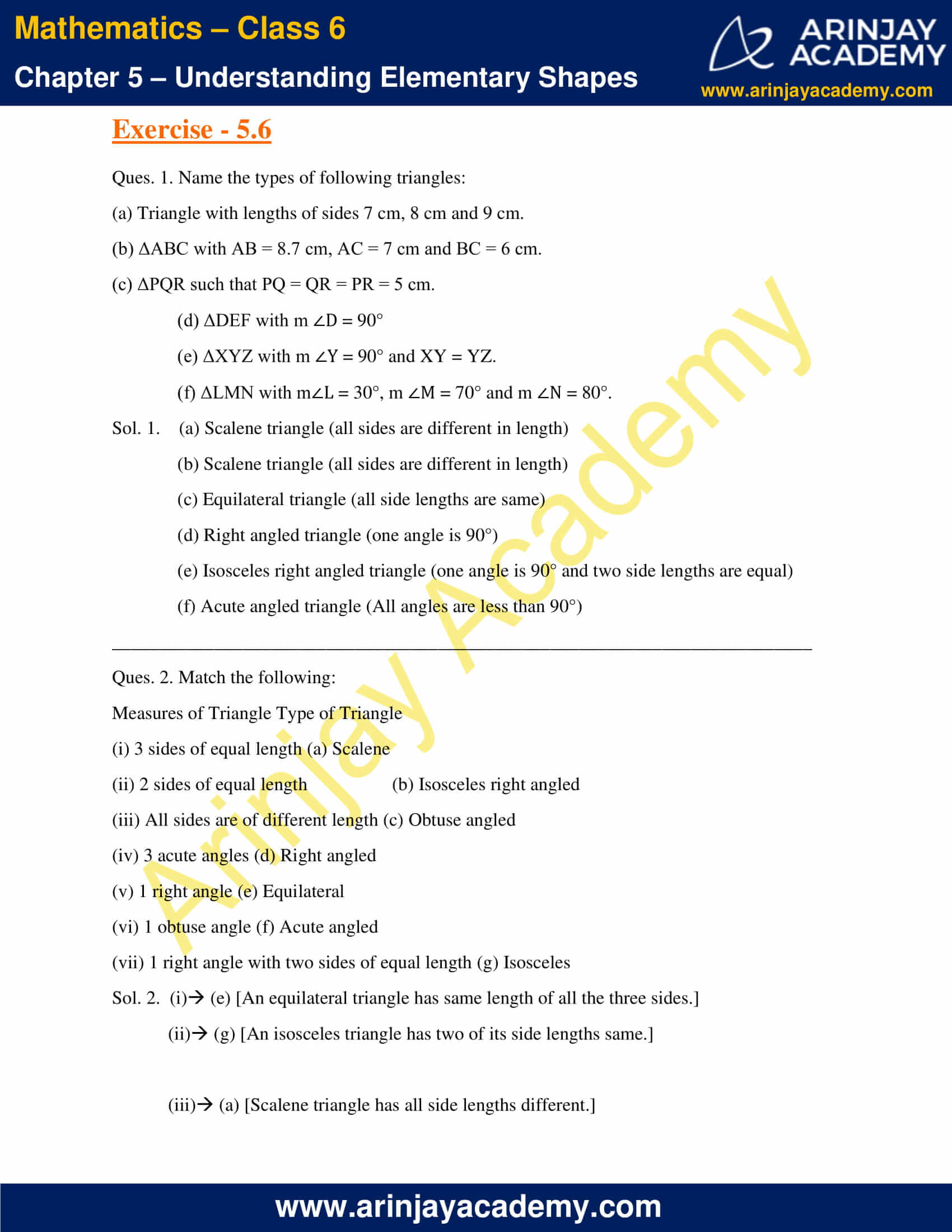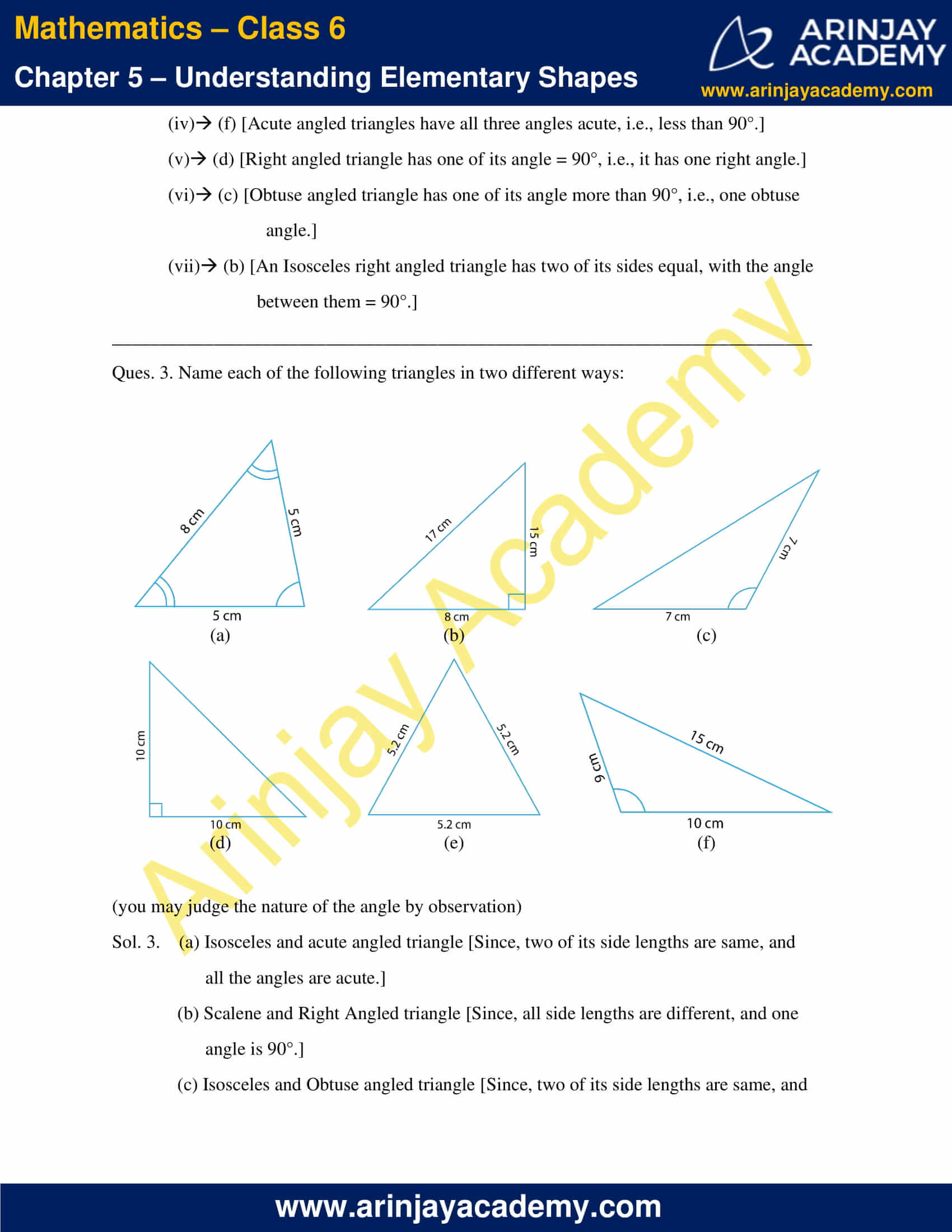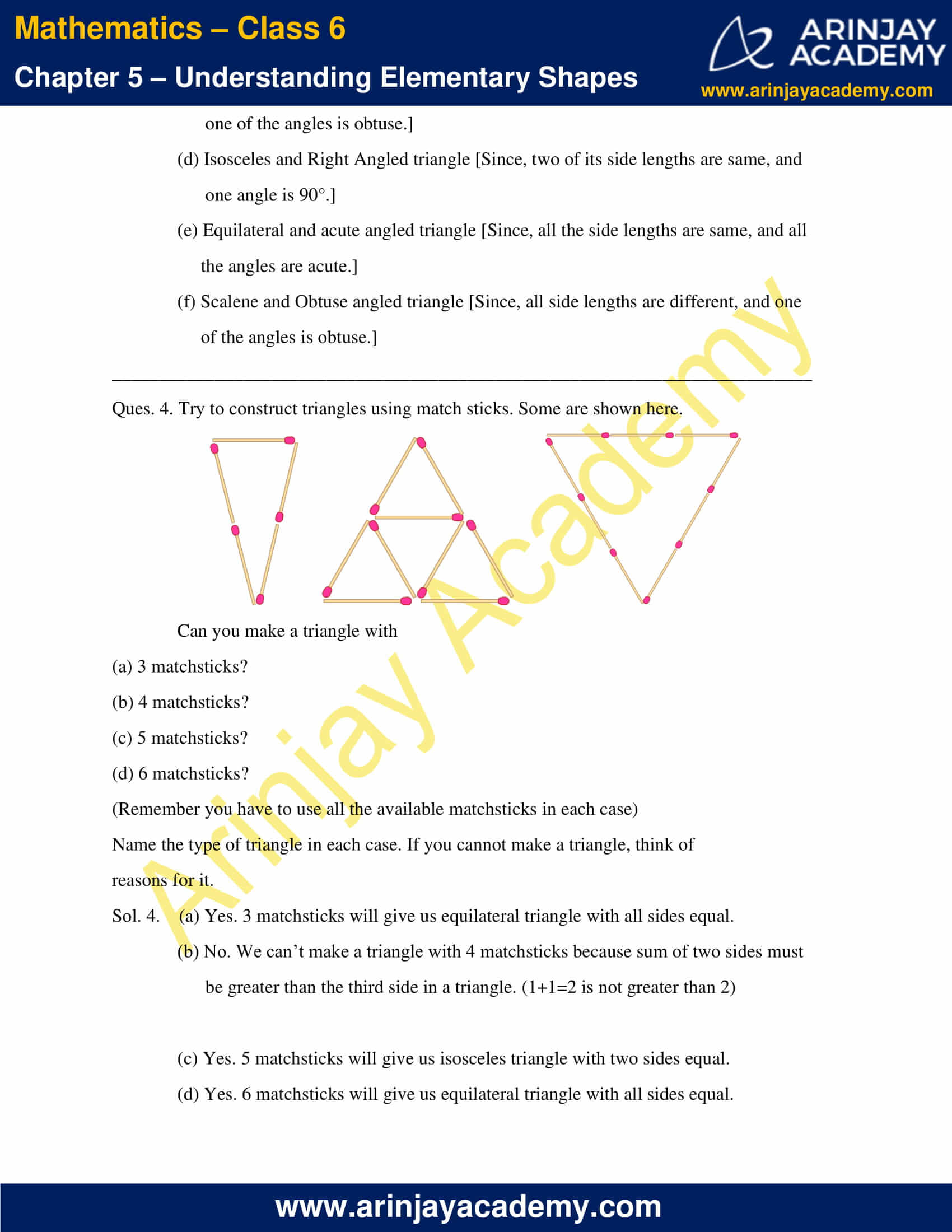### NCERT Solutions for Class 6 Maths Chapter 5 Exercise 5.7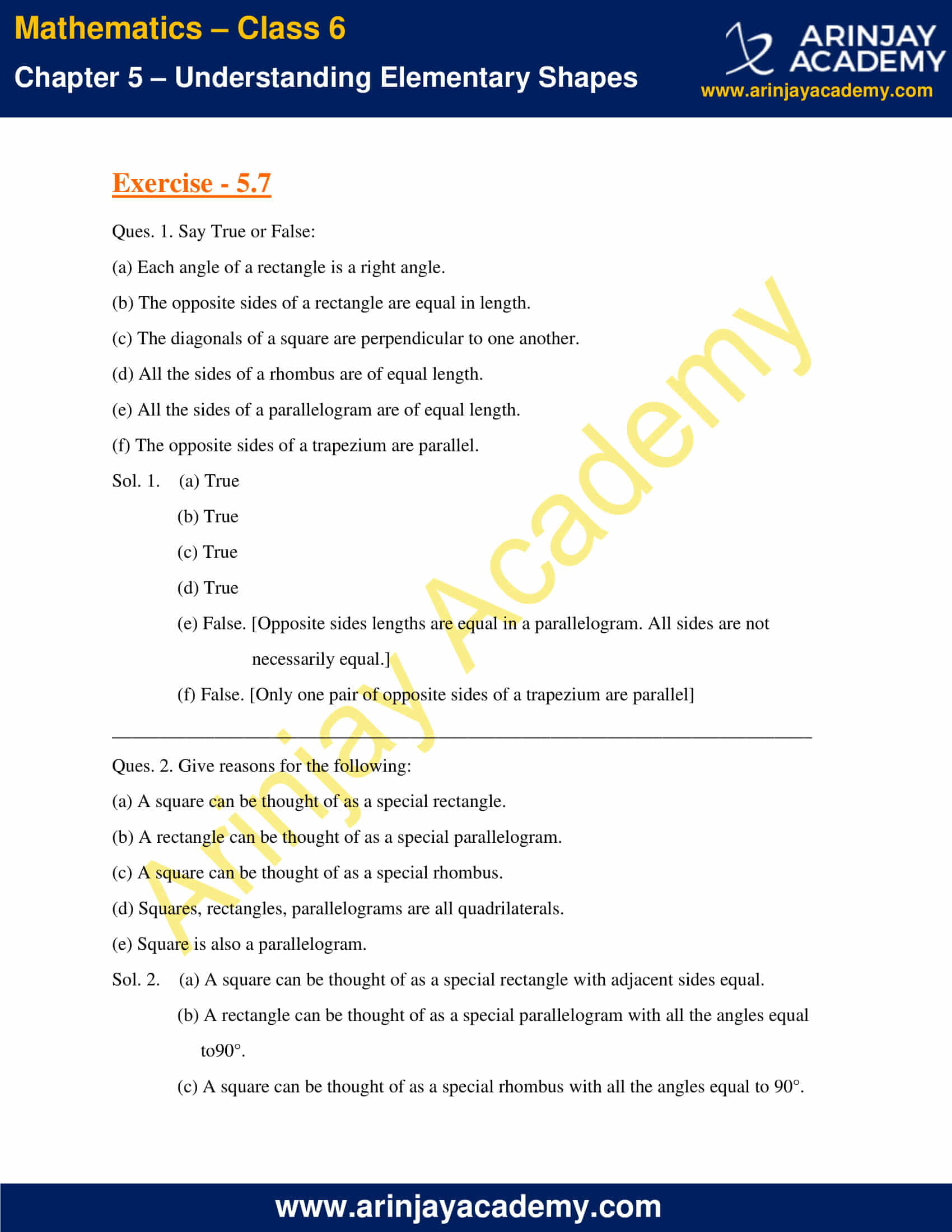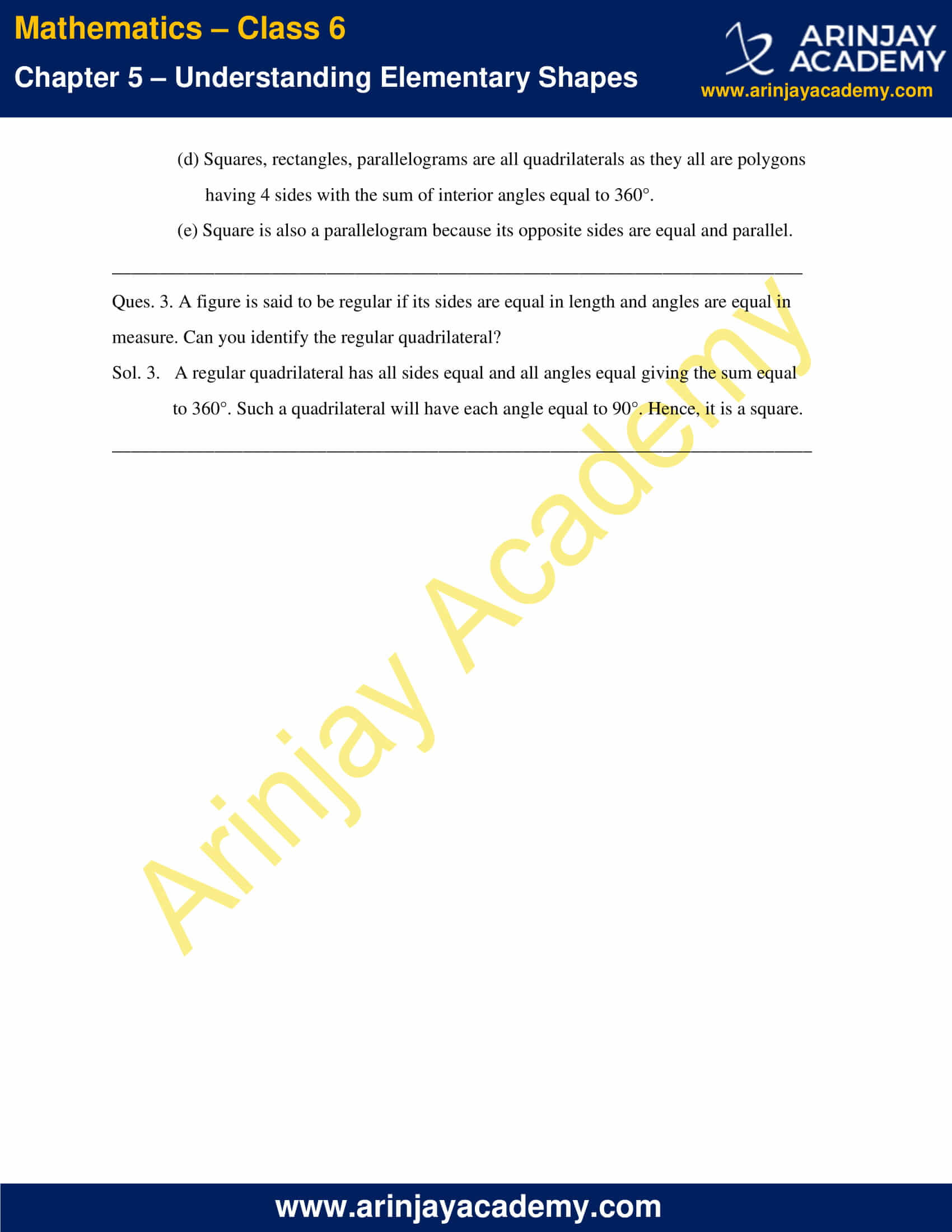### NCERT Solutions for Class 6 Maths Chapter 5 Exercise 5.8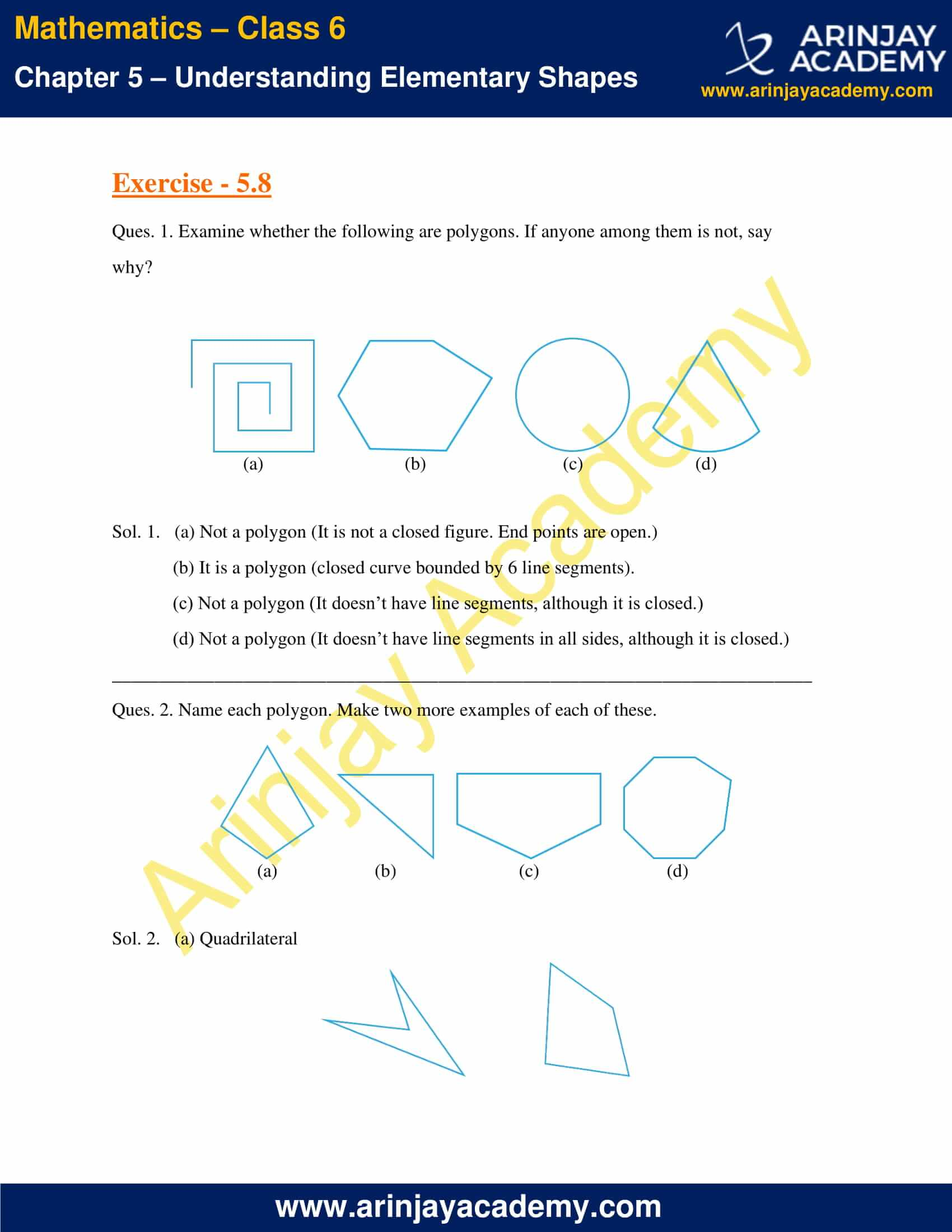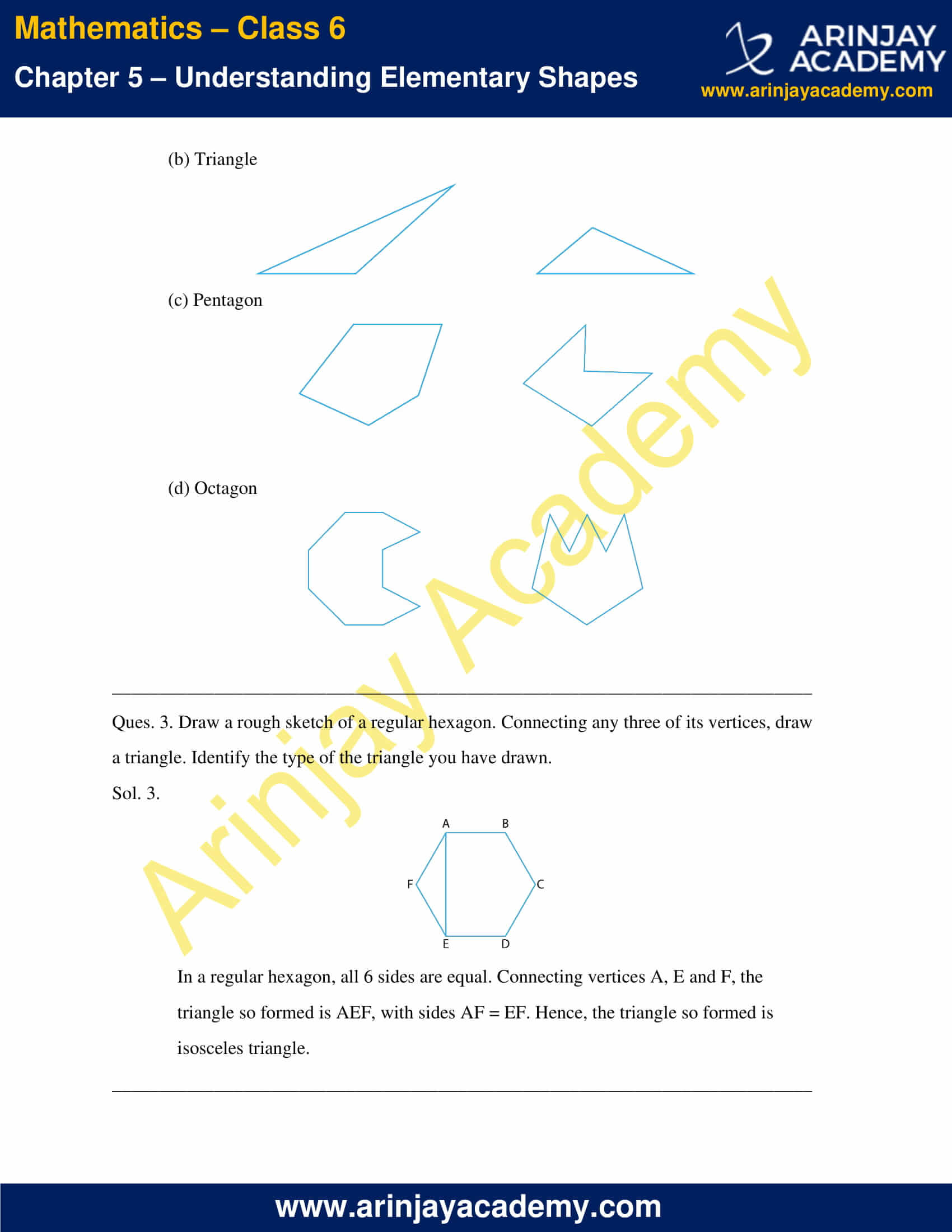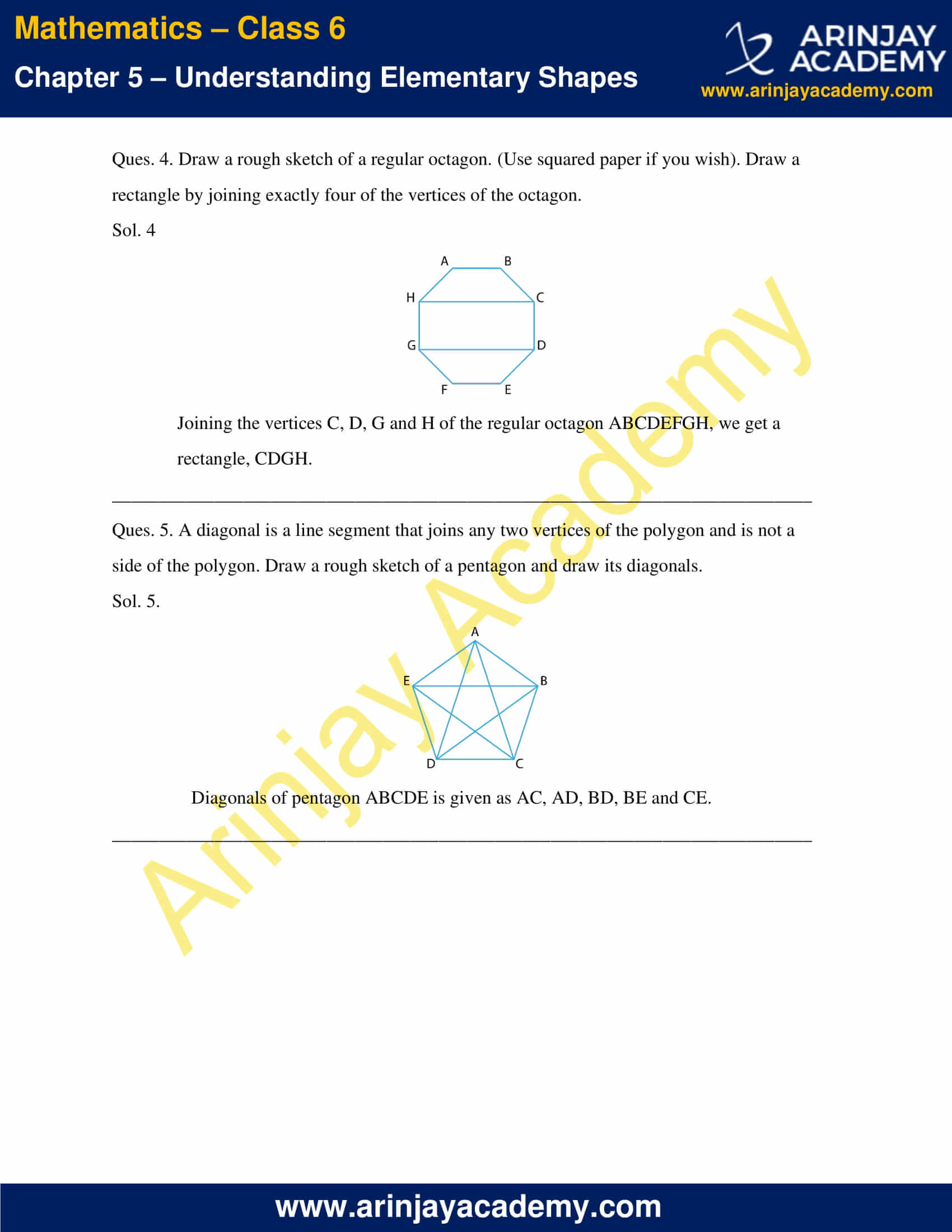### NCERT Solutions for Class 6 Maths Chapter 5 Exercise 5.9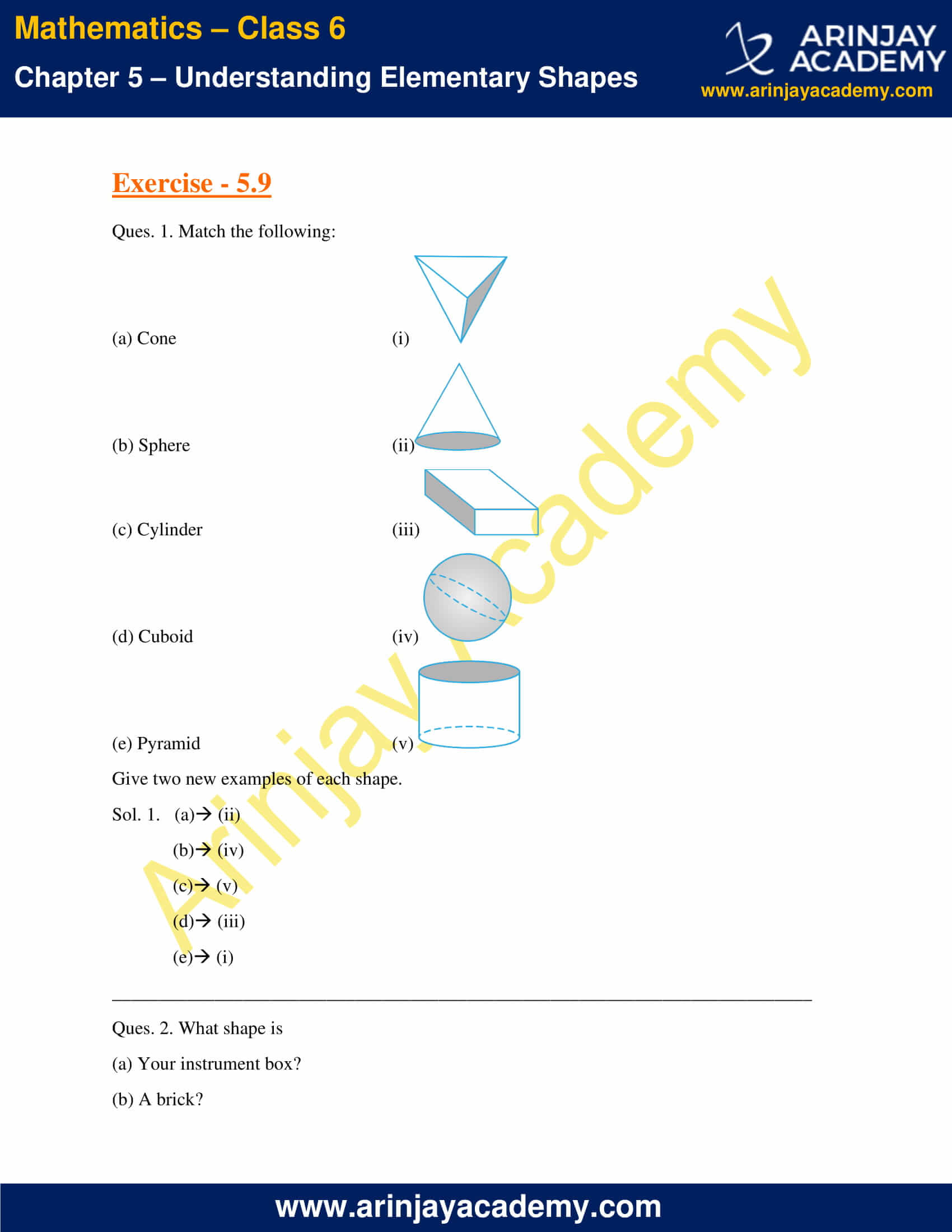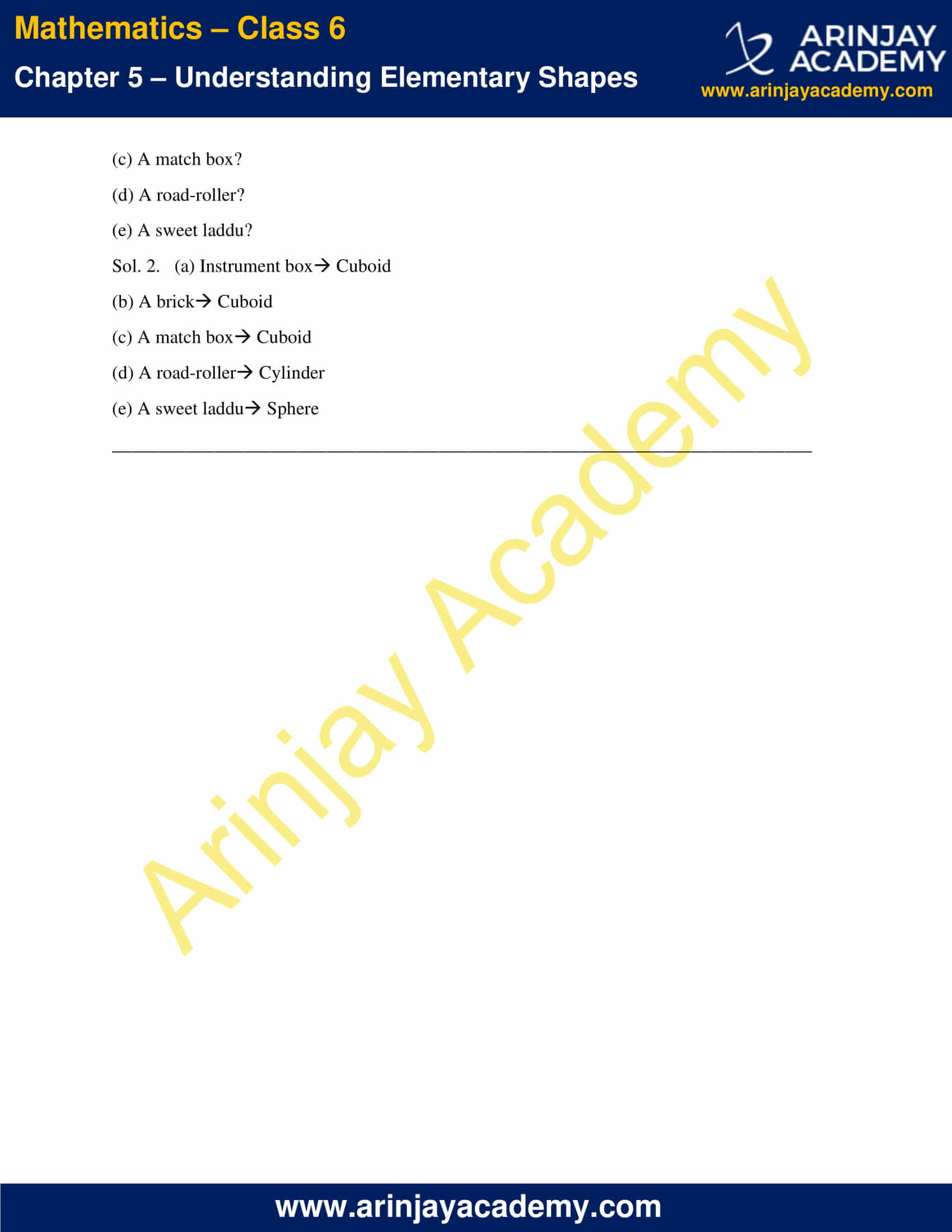### NCERT Solutions for Class 6 Maths Chapter 5 Exercise 5.1

Ques. 1. What is the disadvantage in comparing line segments by mere observation?

Sol. 1. There are a lot of chances of error, due to improper viewing, while comparing line segments by mere observation.

Ques. 2. Why is it better to use a divider than a ruler, while measuring the length of a line segment?

Sol. 2. A divider gives accurate measurement of the length of a line segment as compared to that obtained by a ruler.

Ques. 3. Draw any line segment, say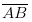. Take any point C lying in between A and B. Measure the lengths of AB, BC and AC. Is AB = AC + CB? [Note: If A,B,C are any three points on a line such that AC + CB = AB, then we can be sure that C lies between A and B.]

Sol. 3.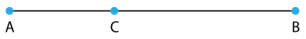Yes, AB = AC + CB. This is because C lies between A and B.

Ques. 4. If A,B,C are three points on a line such that AB = 5 cm, BC = 3 cm and AC = 8 cm, which one of them lies between the other two?

Sol. 4. AC = AB + BC
Since, 8 = 5 + 3
Hence, point B lies between A and C.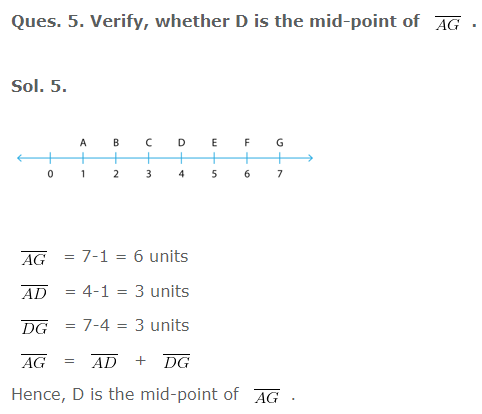Ques. 6. If B is the mid-point of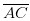and C is the mid-point of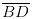where A,B,C,D lie on a straight line, say why AB = CD?

Sol. 6. B is the mid-point of AC, means AB = BC……(1)
C is the mid-point of BD, means BC = CD……(2)
From equation (1) & (2),
AB = BC = CD
Hence, AB = CD.

Ques. 7. Draw five triangles and measure their sides. Check in each case, if the sum of the lengths of any two sides is always less than the third side.

Sol. 7. The following are the five triangles along with their measurements.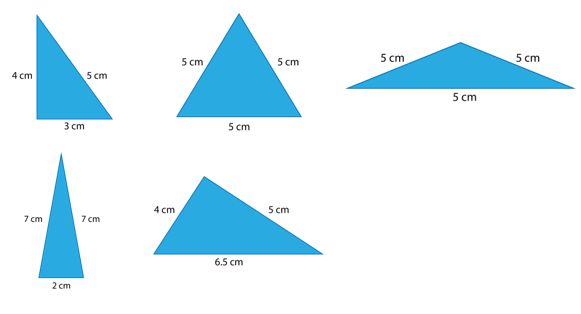In all the above five cases, we can see that the sum of any two sides of a triangle is always greater than the third side.
For example, in the first triangle, 4 + 5 = 9 > 3 or 3 + 4 = 7 > 5 or 5 + 3 = 8 > 4.
Similarly, in all the other triangles, the same rule follows.

### NCERT Solutions for Class 6 Maths Chapter 5 Exercise 5.2

Ques. 1. What fraction of a clockwise revolution does the hour hand of a clock turn through, when it goes from:
(a) 3 to 9
(b) 4 to 7
(c) 7 to 10
(d) 12 to 9
(e) 1 to 10
(f) 6 to 3

Sol. 1.

(a) One revolution of clock, i.e., 12 hours of a clock takes 360 ˚.
So, in one hour, the hour hand of the clock rotate by 360˚/12 = 30˚.
From 3 to 9, i.e., in 6 hours, the hour hand of the clock turns through
30×6=180˚.
Hence, the fraction of a clockwise revolution does the hour hand of a clock turn through, when it goes from 3 to 9 = 180˚/360˚ = 1/2

(b) One revolution of clock, i.e., 12 hours of a clock take 360˚.
So, in one hour, the hour hand of the clock rotate by 360˚/12 = 30˚.
From 4 to 7, i.e., in 3 hours, the hour hand of the clock turns through
30×3 = 90˚.
Hence, the fraction of a clockwise revolution does the hour hand of a clock turn through, when it goes from 4 to 7 = 90˚/360˚ = 1/4

(c) One revolution of clock, i.e., 12 hours of a clock take 360˚.
So, in one hour, the hour hand of the clock rotate by 360˚/12 = 30˚.
From 7 to 10, i.e., in 3 hours, the hour hand of the clock turns through
30×3 = 90˚.
Hence, the fraction of a clockwise revolution does the hour hand of a clock turn through, when it goes from 7 to 10 = 90˚/360˚ = 1/4.

(d) One revolution of clock, i.e., 12 hours of a clock take 360˚.
So, in one hour, the hour hand of the clock rotate by 360˚/12 = 30˚.
From 12 to 9, i.e., in 9 hours, the hour hand of the clock turns through
30×9 = 270˚.
Hence, the fraction of a clockwise revolution does the hour hand of a clock turn through, when it goes from 12 to 9 = 270˚/360˚ = 3/4.

(e) One revolution of clock, i.e., 12 hours of a clock take 360˚.
So, in one hour, the hour hand of the clock rotate by 360/12 = 30˚.
From 1 to 10, i.e., in 9 hours, the hour hand of the clock turns through
30×9 = 270˚.
Hence, the fraction of a clockwise revolution does the hour hand of a clock turn through, when it goes from 1 to 10 = 270˚/360˚ = 3/4.

(f) One revolution of clock, i.e., 12 hours of a clock take 360˚.
So, in one hour, the hour hand of the clock rotate by 360/12 = 30˚.
From 6 to 3, i.e., in 9 hours, the hour hand of the clock turns through
30×9 = 270˚.
Hence, the fraction of a clockwise revolution does the hour hand of a clock turn through, when it goes from 6 to 3 = 270˚/360˚ = 3/4.

Ques. 2. Where will the hand of a clock stop if it
(a) starts at 12 and makes 1/2 of a revolution, clockwise?
(b) starts at 2 and makes 1/2 of a revolution, clockwise?
(c) starts at 5 and makes 1/4 of a revolution, clockwise?
(d) starts at 5 and makes 3/4 of a revolution, clockwise?

Sol. 2. (a) 1/2 of a revolution implies 1/2 of 360˚, i.e., 180˚.
Starting at 12 and making 1/2 of a revolution, clockwise means that, it has turned 180˚ clockwise.
In 1 hour, the hand of the clock takes 30˚.
So, 180˚ can be turned through in 6 hours in clockwise direction.
Hence, the hand of the clock stops at 6 hours clockwise from 12, i.e., at 6.

(b) 1/2 of a revolution implies 1/2 of 360˚, i.e., 180˚.
Starting at 2 and making 1/2 of a revolution, clockwise means that, it has turned 180˚ clockwise.
In 1 hour, the hand of the clock takes 30˚.
So, 180˚ can be turned through in 6 hours in clockwise direction.
Hence, the hand of the clock stops at 6 hours clockwise from 2, i.e., at 8.

(c) 1/4 of a revolution implies 1/4 of 360˚, i.e., 90˚.
Starting at 5 and making 1/4 of a revolution, clockwise means that, it has turned 90˚ clockwise.
In 1 hour, the hand of the clock takes 30˚.
So, 90˚ can be turned through in 3 hours in clockwise direction.
Hence, the hand of the clock stops at 3 hours clockwise from 5, i.e., at 8.

(d) 3/4 of a revolution implies 3/4 of 360˚, i.e., 270˚.
Starting at 5 and making 3/4 of a revolution, clockwise means that, it has turned 270˚ clockwise.
In 1 hour, the hand of the clock takes 30˚.
So, 270˚ can be turned through in 9 hours in clockwise direction.
Hence, the hand of the clock stops at 9 hours clockwise from 5, i.e., at 2.

Ques. 3. Which direction will you face if you start facing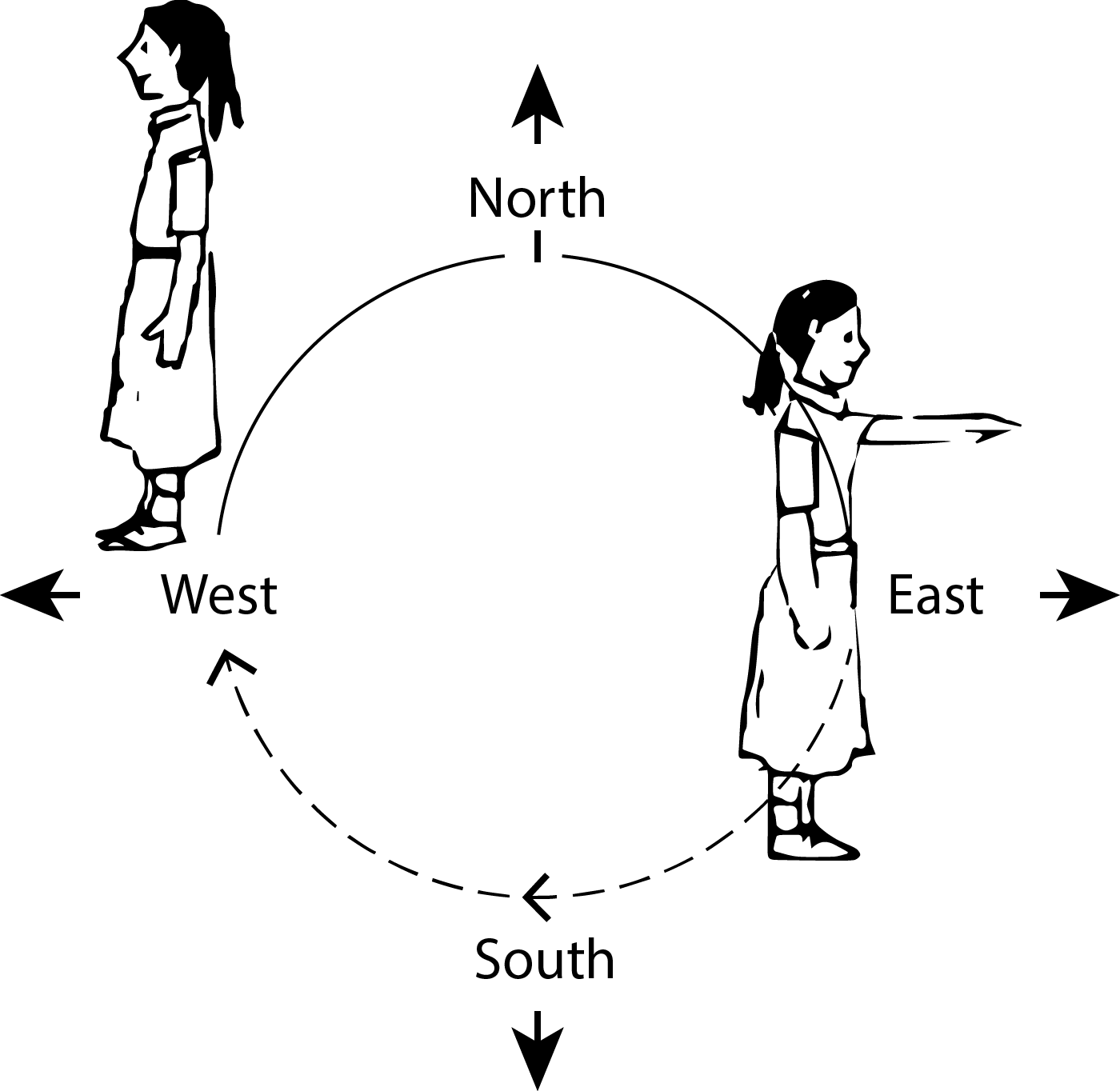(a) east and make 1/2 of a revolution clockwise
(b) east and make 1 1⁄2 of a revolution clockwise?
(c) west and make 3/4 of a revolution anti-clockwise?
(d) south and make one full revolution?
(Should we specify clockwise or anticlockwise for this last question? Why not?)

Sol. 3. (a) Facing east turning 1/2 of a revolution clockwise, i.e., 180˚ clockwise from east, we will face the west direction.

(b) Facing east turning 1 1⁄2 of a revolution clockwise, i.e., 360+180=180˚ clockwise from east, we will face the west direction. (In first 1 revolution we reach the original point. In next 1/2 revolution we turn 180˚ further.)

(c) Facing west turning 3/4 of a revolution anti-clockwise, i.e., 270˚ anti-clockwise from west, we will face the north direction.

(d) Facing south making one full revolution clockwise, or anticlockwise, i.e., 360˚, we will reach the original point of start, i.e., south.

Ques. 4. What part of a revolution have you turned through if you stand facing
(a) east and turn clockwise to face north?
(b) south and turn clockwise to face east?
(c) west and turn clockwise to face east?
Sol. 4.  (a) If we stand facing east and turn clockwise to face north, we turn 3/4 of a revolution.
(b) If we stand facing south and turn clockwise to face east, we turn 3/4 of a revolution.
(c) If we stand facing west and turn clockwise to face east, we turn 1/2 of a revolution.

Ques. 5. Find the number of right angles turned through by the hour hand of a clock when it goes from
(a) 3 to 6
(b) 2 to 8
(c) 5 to 11
(d) 10 to 1
(e) 12 to 9
(f) 12 to 6

Sol. 5. (a) From 3 to 6, i.e., in 3 hours, the hour hand of the clock turns 30˚ ×3=90˚, i.e., 1 right angle.
(b) From 2 to 8, i.e., in 6 hours, the hour hand of the clock turns 30˚ ×6=180˚, i.e., 2 right angles.
(c) From 5 to 11, i.e., in 6 hours, the hour hand of the clock turns 30˚ ×6=180˚, i.e., 2 right angles.
(d) From 10 to 1, i.e., in 3 hours, the hour hand of the clock turns 30˚ ×3=90˚, i.e., 1 right angle.
(e) From 12 to 9, i.e., in 9 hours, the hour hand of the clock turns 30˚ ×9=270˚, i.e., 3 right angles.
(f) From 12 to 6, i.e., in 6 hours, the hour hand of the clock turns 30˚ ×6=180˚, i.e., 2 right angles.

Ques. 6. How many right angles do you make if you start facing
(a) south and turn clockwise to west?
(b) north and turn anti-clockwise to east?
(c) west and turn to west?
(d) south and turn to north?

Sol. 6. (a) Facing south and turning clockwise to west, it takes 90˚, i.e., 1 right angle.
(b) Facing north and turning anti-clockwise to east, it takes 270˚, i.e., 3 right angles.
(c) Facing west and turning to west, it takes 360˚, i.e., 4 right angles.
(d) Facing south and turning to north, it takes 180˚, i.e., 2 right angles.

Ques. 7. Where will the hour hand of a clock stop if it starts
(a) from 6 and turns through 1 right angle?
(b) from 8 and turns through 2 right angles?
(c) from 10 and turns through 3 right angles?
(d) from 7 and turns through 2 straight angles?

Sol. 7. (a) Starting from 6 and turning through 1 right angle, the hour hand of the clock turns 90˚ clockwise from 6, i.e., it stops at 9.
(b) Starting from 8 and turning through 2 right angles, the hour hand of the clock turns 180˚ clockwise from 8, 180/30 i.e.,  = 6 hours ahead. Hence, it stops at 2.
(c) Starting from 10 and turning through 3 right angles, the hour hand of the clock turns 270˚ clockwise from 10, i.e., 270/30 = 9 hours ahead. Hence, it stops at 7.
(d) Starting from 7 and turning through 2 straight angles, the hour hand of the clock turns 2×180=360˚ clockwise (or anticlockwise) from 7, i.e., it stops at 7.

### NCERT Solutions for Class 6 Maths Chapter 5 Exercise 5.3

Ques. 1. Match the following: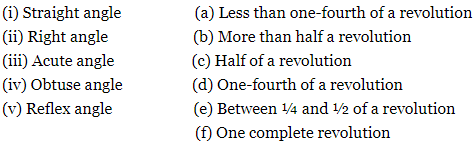Sol. 1. (i) (c) A straight angle is of 180 degree, i.e., half of  a revolution.
(ii) (d) A right angle is of 90 degree which is one-fourth of a revolution.
(iii) ⇒ (a) Acute angle is an angle more than 0 degree and less than 90 degree. Hence it makes less than ¼ of a revolution.
(iv) ⇒ (e) Obtuse angle is an angle more than 90 degree and less than 180 degree.  Hence, it makes between ¼ and ½ of a revolution.
(v) ⇒ (b) More than half of the revolution is a reflex angle.

Ques. 2. Classify each one of the following angles as right, straight, acute, obtuse or reflex :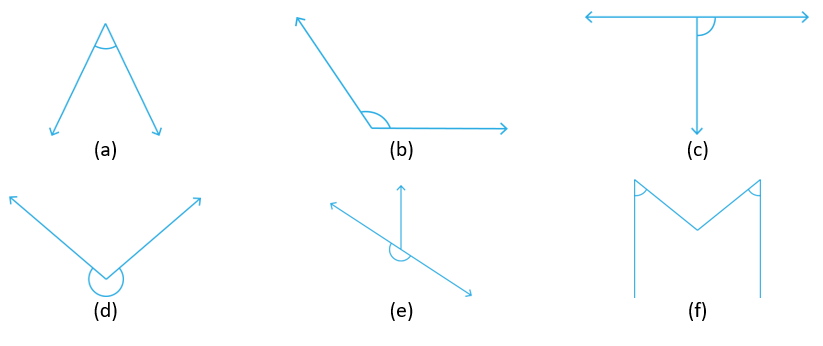Sol. 2. (a) Acute angle (Angle less than 90 degree)
(b) Obtuse angle (Angle more than 90 degree)
(c) Right angle (Angle equal to 90 degree)
(d) Reflex angle (Angle more than 180 degree)
(e) Straight angle (Angle equal to 180 degree)
(f) Acute angle (Angle less than 90 degree)

### NCERT Solutions for Class 6 Maths Chapter 5 Exercise 5.4

Ques. 1. What is the measure of (i) a right angle? (ii) a straight angle?

Sol. 1. (i) A right angle measures 90˚.
(ii) A straight angle measures 180˚.

Ques. 2. Say True or False:
(a) The measure of an acute angle  90°.
(b) The measure of an obtuse angle < 90°.
(c) The measure of a reflex angle > 180°.
(d) The measure of one complete revolution = 360°.
(e) If m ∠A = 53° and m ∠B = 35°, then m ∠A > m ∠B.

Sol. 2. (a) True
(b) False. Obtuse angle measures more than 90˚ and less than 180˚.
(c) True
(d) True
(e) True. (As, 53>35)

Ques. 3. Write down the measures of
(a) some acute angles.
(b) some obtuse angles.
(give at least two examples of each).

Sol. 3. (a) Acute Angle = 64°, 85°
(b) Obtuse Angle = 97°, 164°

Ques. 4. Measure the angles given below using the Protractor and write down the measure.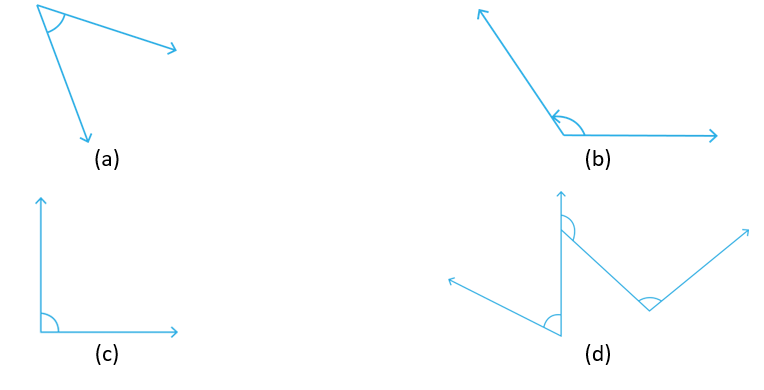Sol. 4.   Upon measuring using protractor, we find out that the measures are:

a) 40° (acute angle)
b) 130° (obtuse angle)
c) 90° (right angle)
d) 60° (acute angle)

___________________________________________________________________________

Ques. 5. Which angle has a large measure? First estimate and then measure.

Measure of Angle A =
Measure of Angle B =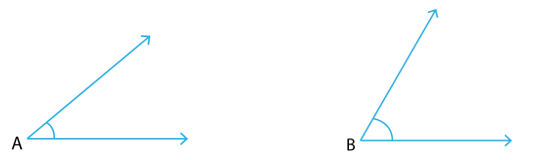Sol. 5. Upon estimating, we can see that Angle B has a larger measure than Angle A.
Upon measuring, we find out, ∠A=40°, ∠B=60°.

Ques. 6. From these two angles which has larger measure? Estimate and then confirm by measuring them.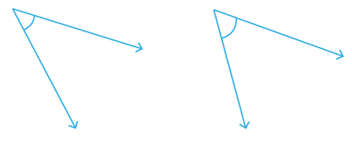Sol. 6. Upon estimating, we can see that the second angle has a larger measure.
Upon measuring, we find out, ∠1=45°, ∠2=55°.

Ques. 7. Fill in the blanks with acute, obtuse, right or straight:
(a) An angle whose measure is less than that of a right angle is______.
(b) An angle whose measure is greater than that of a right angle is ______.
(c) An angle whose measure is the sum of the measures of two right angles is _____.
(d) When the sum of the measures of two angles is that of a right angle, then each one of them is ______.
(e) When the sum of the measures of two angles is that of a straight angle and if one of them is acute then the other should be _______.

Sol. 7. (a) An angle whose measure is less than that of a right angle is acute.
(b) An angle whose measure is greater than that of a right angle is obtuse.
(c) An angle whose measure is the sum of the measures of two right angles is straight.
(d) When the sum of the measures of two angles is that of a right angle, then each one of them is acute.
(e) When the sum of the measures of two angles is that of a straight angle and if one of them is acute then the other should be obtuse.

Ques. 8. Find the measure of the angle shown in each figure. (First estimate with your eyes and then find the actual measure with a protractor).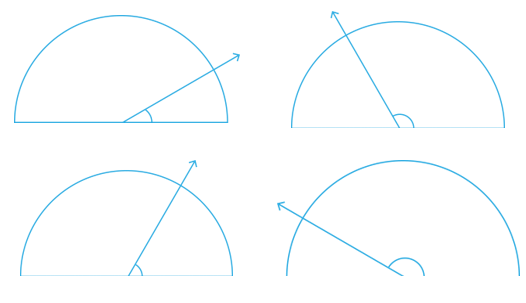Sol. 8. Upon estimating and then measuring we find out that the measurements are:
(i) 30°
(ii) 120°
(iii) 60°
(iv) 150°

Ques. 9. Find the angle measure between the hands of the clock in each figure: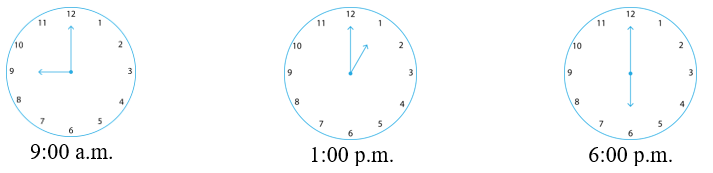Sol. 9.  One complete revolution = 360° (in 12 hours)
Angle subtended in one hour = 360/12 = 30°

(i) Angle between 9 and 12 = (12-9) × 30° = 3×30° = 90°
(ii) Angle between 12 and 1 = 30°
(iii) Angle between 12 and 6 = 6 × 30° = 180°

Ques. 10. Investigate In the given figure, the angle measures 30°. Look at the same figure through a magnifying glass. Does the angle become larger? Does the size of the angle change?

Sol. 10.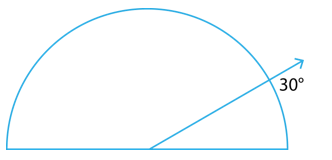No, the angle remains the same.

Ques. 11. Measure and classify each angle: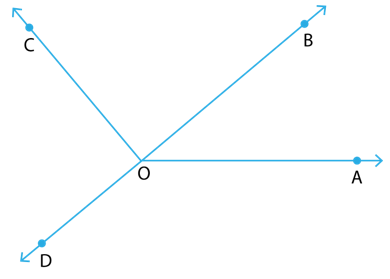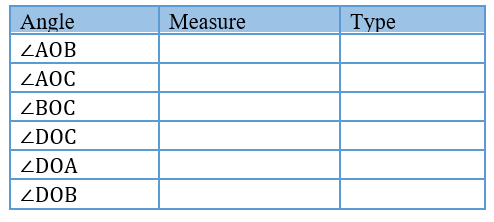Sol. 11.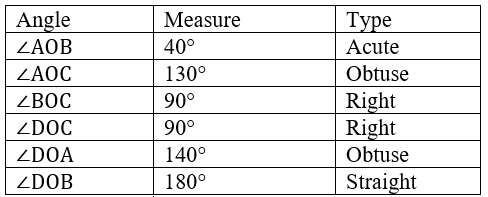### NCERT Solutions for Class 6 Maths Chapter 5 Exercise 5.5

Ques. 1. Which of the following are models for perpendicular lines:
(a) The adjacent edges of a table top.
(b) The lines of a railway track.
(c) The line segments forming the letter ‘L’.
(d) The letter V.

Sol. 1. (a) The adjacent edges of a table top form perpendicular lines.
(b) The lines of a railway track are parallel to each other. So, they don’t form perpendicular lines.
(c) The line segments forming the letter ‘L’ is a model for perpendicular lines.
(d) The letter V forms an acute angle, and hence, does not represent perpendicular line model.
Hence, only (a) and (c) are models for perpendicular lines.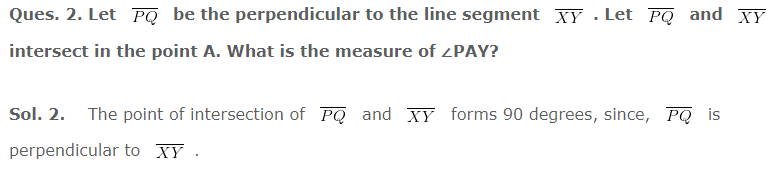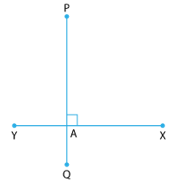Hence, ∠PAY = 90°.

Ques. 3. There are two set-squares in your box. What are the measures of the angles that are formed at their corners? Do they have any angle measure that is common?

Sol. 3. One set square has the measure of 30°, 60° and 90°.
The other one measures 45°, 45° and 90°.
The measure of one angle is common, i.e., 90°.

Ques. 4. Study the diagram. The line l is perpendicular to line m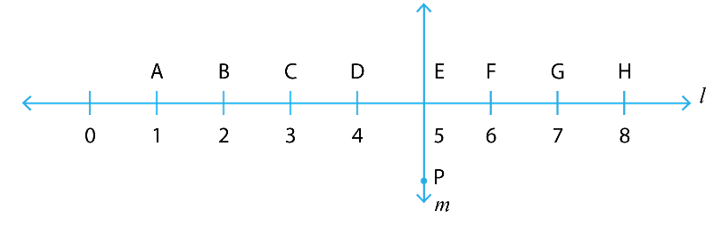(a) Is CE = EG?

(b) Does PE bisect CG?
(c) Identify any two line segments for which PE is the perpendicular bisector.
(d) Are these true? (i) AC > FG        (ii) CD = GH               (iii) BC < EH.

Sol. 4. (a) Yes. CE = EG = 2 units
(b) Yes. PE bisects CG at E, such that CE = EG.
(c)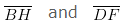are the line segments for which PE is the perpendicular bisector.
(d) (i) True. (AC = 2 units, FG = 1unit. Hence, AC>FG )
(ii) True. (CD = GH = 1unit)
(iii) True. (BC = 1unit, EH = 3 units. Hence, BC<EH)

### NCERT Solutions for Class 6 Maths Chapter 5 Exercise 5.6

Ques. 1. Name the types of following triangles:
(a) Triangle with lengths of sides 7 cm, 8 cm and 9 cm.
(b) ΔABC with AB = 8.7 cm, AC = 7 cm and BC = 6 cm.
(c) ΔPQR such that PQ = QR = PR = 5 cm.
(d) ΔDEF with m ∠D = 90°
(e) ΔXYZ with m ∠Y = 90° and XY = YZ.
(f) ΔLMN with m ∠L = 30°, m ∠M = 70° and m ∠N = 80°.

Sol. 1. (a) Scalene triangle (all sides are different in length)
(b) Scalene triangle (all sides are different in length)
(c) Equilateral triangle (all side lengths are same)
(d) Right angled triangle (one angle is 90°)
(e) Isosceles right angled triangle (one angle is 90° and two side lengths are equal)
(f) Acute angled triangle (All angles are less than 90°)

Ques. 2. Match the following: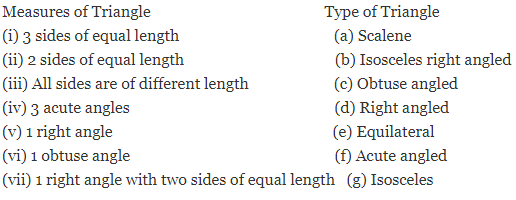Sol. 2.  (i) (e) [An equilateral triangle has same length of all the three sides.]
(ii) (g) [An isosceles triangle has two of its side lengths same.]
(iii) (a) [Scalene triangle has all side lengths different.]
(iv) (f) [Acute angled triangles have all three angles acute, i.e., less than 90°.]
(v) (d) [Right angled triangle has one of its angle = 90°, i.e., it has one right angle.]
(vi) (c) [Obtuse angled triangle has one of its angle more than 90°, i.e., one obtuse angle.]
(vii) (b) [An Isosceles right angled triangle has two of its sides equal, with the angle between them = 90°.]

Ques. 3. Name each of the following triangles in two different ways: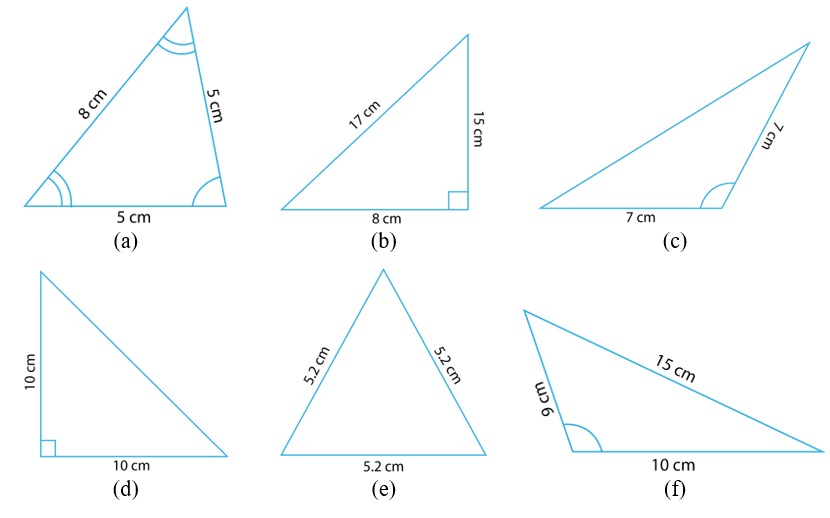(you may judge the nature of the angle by observation)

Sol. 3.  (a) Isosceles and acute angled triangle [Since, two of its side lengths are same, and all the angles are acute.]
(b) Scalene and Right Angled triangle [Since, all side lengths are different, and one angle is 90°.]
(c) Isosceles and Obtuse angled triangle [Since, two of its side lengths are same, and one of the angles is obtuse.]
(d) Isosceles and Right Angled triangle [Since, two of its side lengths are same, and one angle is 90°.]
(e) Equilateral and acute angled triangle [Since, all the side lengths are same, and all the angles are acute.]
(f) Scalene and Obtuse angled triangle [Since, all side lengths are different, and one of the angles is obtuse.]

Ques. 4. Try to construct triangles using match sticks. Some are shown here.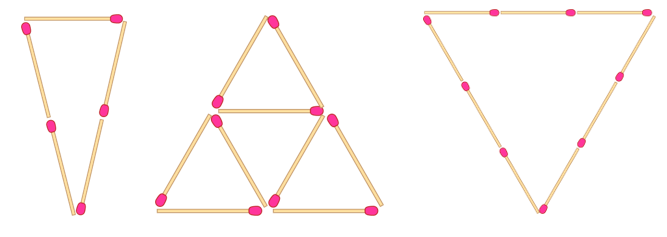Can you make a triangle with
(a) 3 matchsticks?
(b) 4 matchsticks?
(c) 5 matchsticks?
(d) 6 matchsticks?
(Remember you have to use all the available matchsticks in each case)
Name the type of triangle in each case. If you cannot make a triangle, think of reasons for it.

Sol. 4. (a) Yes. 3 matchsticks will give us equilateral triangle with all sides equal.
(b) No. We can’t make a triangle with 4 matchsticks because sum of two sides must be greater than the third side in a triangle. (1+1=2 is not greater than 2)
(c) Yes. 5 matchsticks will give us isosceles triangle with two sides equal.
(d) Yes. 6 matchsticks will give us equilateral triangle with all sides equal.

### NCERT Solutions for Class 6 Maths Chapter 5 Exercise 5.7

Ques. 1. Say True or False:
(a) Each angle of a rectangle is a right angle.
(b) The opposite sides of a rectangle are equal in length.
(c) The diagonals of a square are perpendicular to one another.
(d) All the sides of a rhombus are of equal length.
(e) All the sides of a parallelogram are of equal length.
(f) The opposite sides of a trapezium are parallel.

Sol. 1. (a) True
(b) True
(c) True
(d) True
(e) False. [Opposite sides lengths are equal in a parallelogram. All sides are not necessarily equal.]
(f) False. [Only one pair of opposite sides of a trapezium are parallel]

Ques. 2. Give reasons for the following:
(a) A square can be thought of as a special rectangle.
(b) A rectangle can be thought of as a special parallelogram.
(c) A square can be thought of as a special rhombus.
(d) Squares, rectangles, parallelograms are all quadrilaterals.
(e) Square is also a parallelogram.

Sol. 2. (a) A square can be thought of as a special rectangle with adjacent sides equal.
(b) A rectangle can be thought of as a special parallelogram with all the angles equal to 90°.
(c) A square can be thought of as a special rhombus with all the angles equal to 90°.
(d) Squares, rectangles, parallelograms are all quadrilaterals as they all are polygons having 4 sides with the sum of interior angles equal to 360°.
(e) Square is also a parallelogram because its opposite sides are equal and parallel.

Ques. 3. A figure is said to be regular if its sides are equal in length and angles are equal in measure. Can you identify the regular quadrilateral?

Sol. 3.   A regular quadrilateral has all sides equal and all angles equal giving the sum equal to 360°. Such a quadrilateral will have each angle equal to 90°. Hence, it is a square.

### NCERT Solutions for Class 6 Maths Chapter 5 Exercise 5.8

Ques. 1. Examine whether the following are polygons. If anyone among them is not, say why?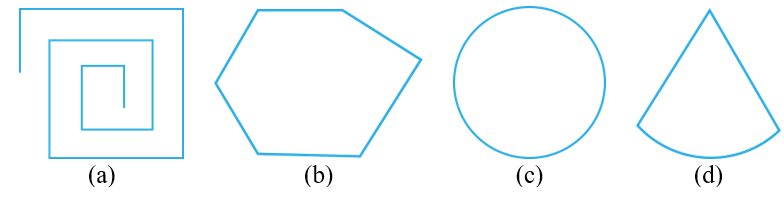Sol. 1. (a) Not a polygon (It is not a closed figure. End points are open.)
(b) It is a polygon (closed curve bounded by 6 line segments).
(c) Not a polygon (It doesn’t have line segments, although it is closed.)
(d) Not a polygon (It doesn’t have line segments in all sides, although it is closed.)

Ques. 2. Name each polygon. Make two more examples of each of these.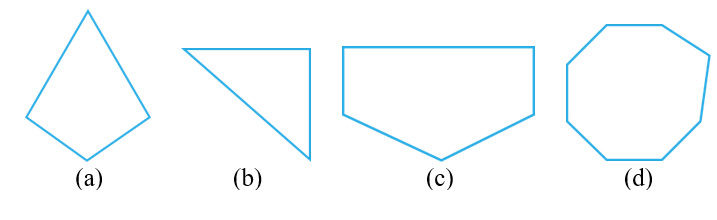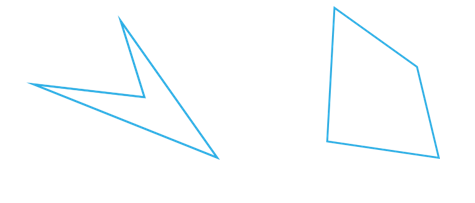(b) Triangle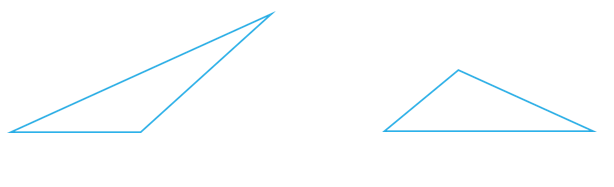(c) Pentagon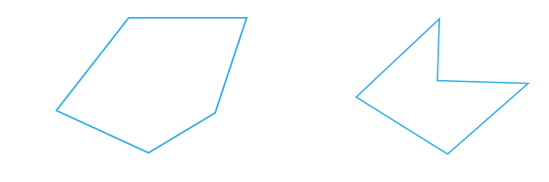(d) Octagon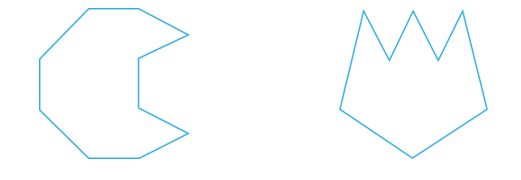Ques. 3. Draw a rough sketch of a regular hexagon. Connecting any three of its vertices, draw a triangle. Identify the type of the triangle you have drawn.

Sol. 3.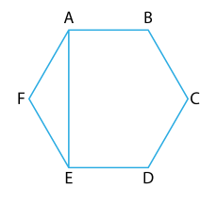In a regular hexagon, all 6 sides are equal. Connecting vertices A, E and F, the triangle so formed is AEF, with sides AF = EF. Hence, the triangle so formed is isosceles triangle.

Ques. 4. Draw a rough sketch of a regular octagon. (Use squared paper if you wish). Draw a rectangle by joining exactly four of the vertices of the octagon.

Sol. 4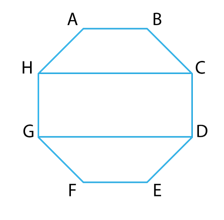Joining the vertices C, D, G and H of the regular octagon ABCDEFGH, we get a rectangle, CDGH.

Ques. 5. A diagonal is a line segment that joins any two vertices of the polygon and is not a side of the polygon. Draw a rough sketch of a pentagon and draw its diagonals.

Sol. 5.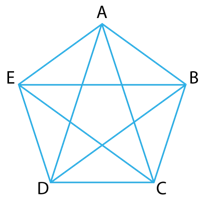Diagonals of pentagon ABCDE is given as AC, AD, BD, BE and CE.

### NCERT Solutions for Class 6 Maths Chapter 5 Exercise 5.9

Ques. 1. Match the following: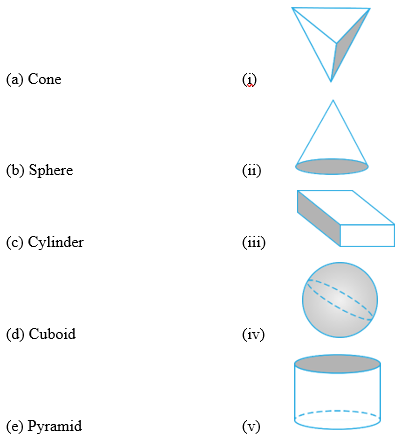Give two new examples of each shape.

Sol. 1. (a) (ii)
(b) (iv)
(c) (v)
(d) (iii)
(e) (i)

Ques. 2. What shape is